# KCET 2019 Maths Paper with Solutions

KCET 2019 Maths question paper with solutions and answer key are available on this page. Students will benefit from the step by step solutions prepared by our subject matter experts and gain a better understanding of the KCET level Maths problems and how to solve them. Students can further practise solving these questions and also check the correct solutions for them. Ultimately, students can improve their speed and accuracy, gain knowledge about the question pattern, types, weightage of marks and duration of the paper. The question paper along with the solutions can be downloaded in the form of PDF below.

## KCET 2019 Maths Question Paper with Solutions

KCET 2019 – Maths
Question 1: If
$$\begin{array}{l}\sqrt{y}\sqrt{x}=\sqrt{(x+y)^{5}}\end{array}$$
, then dy/dx equal to:

a. x/y
b. x+y
c. x-y
d. y/x

$$\begin{array}{l}\sqrt{y}\sqrt{x}=\sqrt{(x+y)^{5}}\end{array}$$

Apply log on both sides

log (y1/3x1/2) = log(x+y)5/6

(1/3) log y+(1/2) log x = (5/6) log(x+y)

(1/3y)(dy/dx)+(1/2x) = (5/6(x+y))(1+dy/dx)

(1/3y)(dy/dx)- (5/6(x+y))dy/dx =(5/6(x+y)) -(1/2x)

(dy/dx)[(1/3y)-(5/6(x+y)] = 5x-3(x+y)/6x(x+y)

(dy/dx) = [(2x-3y)/6x(x+y)][6y(x+y)/(2x-3y)]

dy/dx = y/x

Question 2: Rolle’s theorem is not applicable in which one of the following cases?

a. f(x) = x2-4x+5 in [1, 3]
b. f(x) = x2-x in [0, 1]
c. f(x) = x in [-2, 2]
d. f(x) = [x] in [2.5, 2.7]

Check option:

Option (a):

(1) f(x) is continuous function in [1, 3]

(2) f(x) is differentiable in (1, 3)

Option (b):

(1) f(x) is continuous function in [0, 1]

(2) f(x) is differentiable in (0, 1)

Option (c):

(1) f(x) is continuous function in [–2, 2]

(2) f(x) is not differentiable at point x = 0 in (–2, 2)

Option (d):

(1) f(x) is continuous function in [2.5, 2.7]

(2) f(x) is differentiable in (2.5, 2.7)

Question 3: The interval in which the function f(x) = x3-6x2+9x+10 is increasing in

a. (-∞,1) ⋃ (3, ∞)
b. (-∞,-1) ⋃ (-3, ∞)
c. [1, 3]
d. (-∞,-1) ⋃ [3, ∞)

f(x) = x3-6x2+9x+10

Differentiate with respect to x

f’(x) = 3x2 -12x+9

f’(x) ≥ 0

⇒ 3x2-12x+9 ≥0

⇒ x2-4x+3 ≥ 0

⇒ x2-3x -x+3 ≥ 0

⇒ (x-3) (x-1) ≥ 0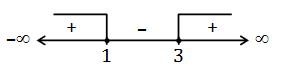x ∈ (-∞, 1] ⋃[3, ∞ )

Question 4: The side of an equilateral triangle are increasing at the rate of 4cm/sec. The rate at which its area is increasing, when the side is 14 cm

a. 10√3 cm2/sec
b. 14√3 cm2/sec
c. 42 cm2/sec
d. 14 cm2/sec

Bonus

Question 5: The value of √24.99 is

a. 4.999
b. 4.899
c. 5.001
d. 4.897

f(x) = √x

⇒ dy/dx = 1/2√x

∆y = (dy/dx) ∆x …(i)

Here x = 25 and ∆x = 0.1

∆y = √x-√(x-∆x)

(dy/dx) ∆x = √25-√(25-0.1)

√24.99 = 5-(1/2×5)×0.1

= 5-0.01

= 4.99

Question 6: If 3x-5≤2 then

a. -1≤x≤7/3
b. 1≤x≤7/3
c. 1≤x≤9/3
d. -1≤x≤9/3

|3x-5| ≤ 2

⇒ -2 ≤3x–5 ≤ 2

⇒ 3 ≤ 3x ≤ 7

⇒ 1 ≤ x ≤7/3

Question 7: A random variable ‘X’ has the following probability distribution:

 X 1 2 3 4 5 6 7 P(X) k-1 3k k 3k 3k2 k2 k2+k

a. 1/5
b. -2
c. 2/7
d. 1/10

$$\begin{array}{l}\sum P(K)=1\end{array}$$

⇒ K-1+3K+K+3K+3K2+K2+K2+K = 1

⇒ 5K2+9K-2 = 0

⇒5K2+10K-K-2 = 0

⇒ (5K-1)(K+2) = 0

5K-1 = 0 or K+2 = 0

K = 1/5 or K = -2 (not possible)

Question 8: If A and B are two events of a sample space S such that P(A) =0.2, P(B) =0.6 and P(AB)=0.5 then P(A’|B)=

a. 3/10
b. 2/3
c. 1/2
d. 1/3

Given that

P(A) = 0.2

P(B) = 0.6

P(A|B) = 0.5

Now

P(A’|B) = 1-P(A|B)

= 1-0.5

= 0.5

= 1/2

Question 9: If ‘X’ has a binomial distribution with parameters n = 6, p and P(X = 2) = 12, P(X = 3) = 5 then P=

a. 5/12
b. 16/21
c. ½
d. 5/16

Bonus

*G indicates one grace mark

Question 10: A man speaks truth 2 out of 3 times. He picks one of the natural numbers in the set S = {1, 2, 3, 4, 5, 6, 7} and reports that it is even. The probability that it is actually even is

a. 2/5
b. 1/5
c. 1/10
d. 3/5

S = {1,2,3,4,5,6,7}

Total probability = 7

P(S1) = Probability of even number = 3/7

P(S2) = Probability of odd number = 4/7

P(ES1) = 2/3

P(ES2) = 1-2/3 = 1/3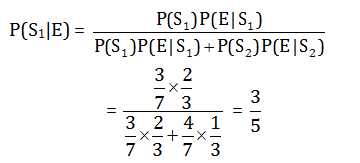Question 11: If U is the universal set with 100 elements; A and B are two sets such that n(A) = 50, n(B) = 60, n(A⋂B) = 20 then n(A’⋂B’) = ?

a. 40
b. 20
c. 90
d. 10

Given that

n(A) = 50

n(B) = 60

n(A⋂B) = 20

Now

n(A’⋂ B’) = P(A⋂B)’

= 100-P(A⋂B)

= 100-[P(A)+P(B)- P(A⋂B)]

= 100-[50+60-20]

= 100-90

= 10

Question 12: The domain of the function f:R→R defined by f(x) = √(x2-x+12)

a. (-∞,3] ⋃[4, ∞)
b. (-∞,3] ⋃(4, ∞)
c. (-∞,3] ⋂ [4, ∞)
d. (3,4)

f(x) = √(x2-7x+12)

Now

x2-7x+12≥0

x2-4x-3x+12≥0

(x-4)(x-3) ≥0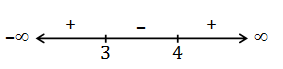x∈(-∞,3] ⋃[4, ∞)

Question 13: If cos x = sin x then, the general solution is

a. x = nπ ± π/4, n∈Z
b. x = 2nπ ± π/4, n∈Z
c. x = nπ+(-1)n π/4, n∈Z
d. x = (2n+1)π ± π/4, n∈Z

cos x = |sinx|

Case-1:

cos x = sin x for x∈ [0, π ]

tan x = 1 for x = π/4

Case-2:

s x = -sin x for x ∈ [π, 2π]

tan x = -1 for x∈ 7π/4

Combining both the cases, we get the general is solution 2nπ±π/4

Question 14: √3 cosec 200– sec 200 =

a. 2
b. 3
c. 4
d. 1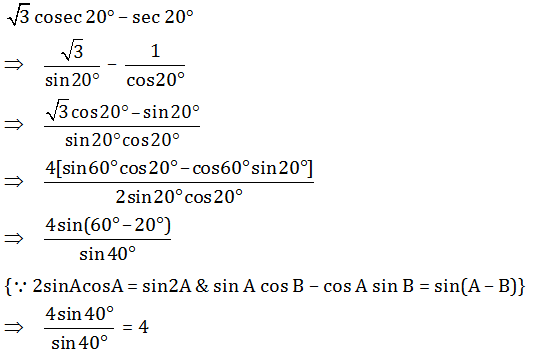Question 15: If P(n): 2n <n! then the smallest positive integer for which P(n) is true, is

a. 2
b. 3
c. 4
d. 5

P(n): 2n< n!

Since

P(1): 2 < 1 is false.

P(2): 22< 2! is false.

P(3): 23< 3! is false.

P(4): 24< 4! is true.

P(5): 25< 5! is true.

So, the smallest positive integer is 4.

Question 16: Foot of the perpendicular drawn from the point (1,3,4) to the plane 2x-y+z+3=0 is

a. (-1,4,3)
b. (0,-4,-7)
c. (1,2,-3)
d. (-3,5,2)

The Dr’s of PA are

x1-1, y1-3, z1-4

The Dr’s n are 2, -1, 1

The Dr’s of PA and n are parallel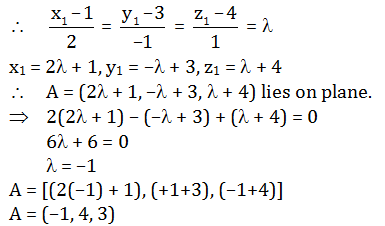Question 17: Acute angle between the line (x-5)/2 = (y+1)/-1 = (z+4)/1 and the plane 3x-4y-z+5 = 0 is:

a. cos-1(9/√364)
b. sin-1(9/√364)
c. cos-1(5/2√13)
d. sin-1(5/2√13)

L: (x-5)/2 = (y+1)/-1 = (z+4)/1

Dr’s of line: 2, -1, 1

P: 3x-4y-z + 5 = 0

Dr’s of normal to plane: -3, 4, 1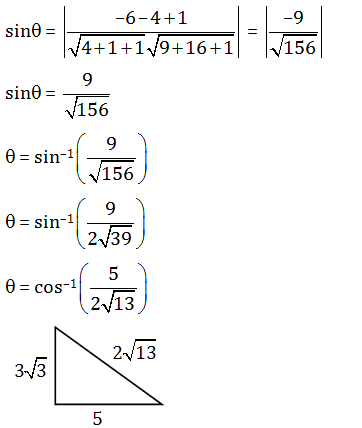Question 18: The distance of the point (1,2,1) form the line (x-1)/2 = (y-2)/1 = (z-3)/2 is

a. 2√3/5
b. 2√5/3
c. √5/3
d. 20/3

Let L: (x-1)/2 = (y-2)/1 = (z-3)/2 = λ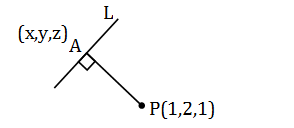$$\begin{array}{l}\overrightarrow{PA}\end{array}$$
= Position vector of A – position of vector of P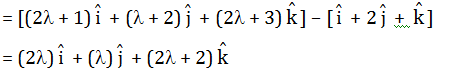Vector PA perpendicular to L.

So

$$\begin{array}{l}\overrightarrow{PA}.L=0\end{array}$$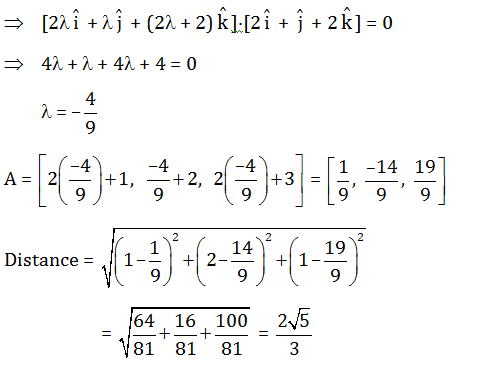Question 19: XY-plane divides the line joining the points A(2,3,-5) and B(-1,-2,-3) in the ratio

a. 2:1 internally
b. 3:2 externally
c. 5:3 internally
d. 5:3 externally

A(2,3,-5), B(-1,-2,-3)

The ratio that xy plane divide line joining the points (x1,y1,z1) and (x2,y2,z2) = -z1:z2

If result is positive, it divides internally otherwise externally.

The ratio that xy plane divides the points (2,3,-5) and (-1,-2,-3)

= -(-5) :(-3)

= -5 : 3

So, 5 : 3 externally in the ratio.

Question 20: The shaded region in the figure is the solution set of the inequations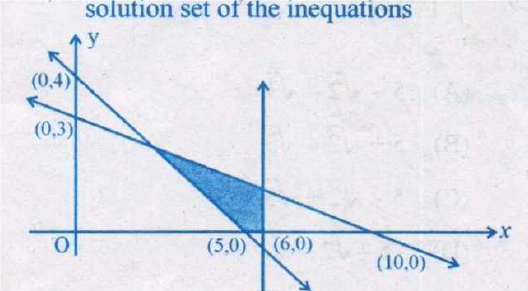a. 4x+5y≥20, 3x+10y≤30, x≤6, x, y≥0.
b. 4x+5y≥20, 3x+10y≤30, x≥6, x, y≥0.
c. 4x+5y≤20, 3x+10y≤30, x≤6, x, y≥0.
d. 4x+5y≤20, 3x+10y≤30, x≥6, x, y≥0.

(1) Since the region lies above the x-axis

So y ≥0

(2) Shaded region is on right side of the y-axis

So x ≥0

(3) Equation of line passing through (5,0) and (0, 4) is

(y-0) = (4/-5) (x-5)

-5y = 4x- 20

4x+5y = 20

(4) Equation of line passing through (10,0) and (0, 3) is

(y-0) = (-3/10) (x-10)

-10y = 3x-30

3x+10y = 30

(5) Shaded region is on left of x = 6.

So x ≤6

Consider the figure and observe the shaded region the inequalities can be written as

x ≥ 0,

y ≥ 0,

x ≤ 6

4x+5y ≥20,

3x+10y ≤ 30

Question 21: The order of the differential equation is

$$\begin{array}{l}y= C_{1}e^{C_{2}+x}+C_{3}e^{C_{4}+x}\end{array}$$

a. 1
b. 2
c. 3
d. 4

$$\begin{array}{l}y= C_{1}e^{C_{2}+x}+C_{3}e^{C_{4}+x}\end{array}$$

Differentiate with respect to x

dy/dx =

$$\begin{array}{l}C_{1}e^{C_{2}+x}+C_{3}e^{C_{4}+x}\end{array}$$

dy/dx = y

(dy/dx)-y = 0

Hence the order is 1.

Question 22: If

$$\begin{array}{l}\left | \vec{a} \right |=16\end{array}$$
,
$$\begin{array}{l}\left | \vec{b} \right |=4\end{array}$$
, then
$$\begin{array}{l}\sqrt{\left | \vec{a\times \vec{b}} \right |^{2}+\left | \vec{a}.\vec{b} \right |^{2}}\end{array}$$

a. 4
b. 8
c. 16
d. 64

$$\begin{array}{l}\left | \vec{a} \right |=16\end{array}$$
$$\begin{array}{l}\left | \vec{b} \right |=4\end{array}$$

Now,

$$\begin{array}{l}\sqrt{\left | \vec{a\times \vec{b}} \right |^{2}+\left | \vec{a}.\vec{b} \right |^{2}}= \sqrt{\left | \vec{a} \right |^{2}\left | \vec{b} \right |^{2}\sin ^{2}\theta +\left | \vec{a} \right |^{2}\left | \vec{b} \right |^{2}\cos ^{2}\theta}\end{array}$$

=

$$\begin{array}{l}\left | \vec{a} \right |\left | \vec{b} \right |\sqrt{\sin ^{2}\theta +\cos ^{2}\theta }\end{array}$$

= 16×4×1

= 64

Question 23: If the angle between

$$\begin{array}{l}\vec{a}\end{array}$$
and
$$\begin{array}{l}\vec{b}\end{array}$$
is
$$\begin{array}{l}\frac{2\pi }{3}\end{array}$$
and the projection of
$$\begin{array}{l}\vec{a}\end{array}$$
in the direction of
$$\begin{array}{l}\vec{b}\end{array}$$
is -2, then
$$\begin{array}{l}\left | \vec{a} \right |\end{array}$$
=

a. 4
b. 3
c. 2
d. 1

Given angle between

$$\begin{array}{l}\vec{a}\end{array}$$
and
$$\begin{array}{l}\vec{b}\end{array}$$
is
$$\begin{array}{l}\frac{2\pi }{3}\end{array}$$

And

$$\begin{array}{l}\frac{\vec{a}.\vec{b}}{\left | \vec{b} \right |}=-2\end{array}$$

$$\begin{array}{l}\frac{\left | \vec{a} \right |\left | \vec{b} \right |\cos \theta }{\left | \vec{b} \right |}=-2\end{array}$$

$$\begin{array}{l}\left | \vec{a} \right |\cos \frac{2\pi }{3}=-2\end{array}$$

$$\begin{array}{l}\left | \vec{a} \right |\times \frac{-1}{2}=-2\end{array}$$

$$\begin{array}{l}\left | \vec{a} \right |=4\end{array}$$

Question 24: A unit vector perpendicular to the plane containing the vectors

$$\begin{array}{l}\hat{i}+2\hat{j}+\hat{k}\end{array}$$
and
$$\begin{array}{l}-2\hat{i}+\hat{j}+3\hat{k}\end{array}$$
is:

a.

$$\begin{array}{l}\frac{\hat{i}+\hat{j}+\hat{k}}{\sqrt{3}}\end{array}$$

b.
$$\begin{array}{l}\frac{\hat{i}+\hat{j}-\hat{k}}{\sqrt{3}}\end{array}$$

c.
$$\begin{array}{l}\frac{-\hat{i}+\hat{j}-\hat{k}}{\sqrt{3}}\end{array}$$

d.
$$\begin{array}{l}\frac{-\hat{i}-\hat{j}-\hat{k}}{\sqrt{3}}\end{array}$$

The vector which is perpendicular to two vectors is given by the cross product of the two vectors.

$$\begin{array}{l}\vec{P}\times \vec{Q}=(-2\hat{i}+\hat{j}+3\hat{k})\times (\hat{i}+2\hat{j}+\hat{k})\end{array}$$

=

$$\begin{array}{l}-5\hat{i}+5\hat{j}-5\hat{k}\end{array}$$
$$\begin{array}{l}\left | \vec{P} \times \vec{Q}\right |=\sqrt{5^{2}+5^{2}+5^{2}}=5\sqrt{3}\end{array}$$

Unit vector perpendicular to P and Q is given by

$$\begin{array}{l}C=\frac{\vec{P}\times \vec{Q}}{\left | \vec{P}\times \vec{Q} \right |}\end{array}$$

=

$$\begin{array}{l}\frac{-5\hat{i}+5\hat{j}-5\hat{k}}{5\sqrt{3}}\end{array}$$

=

$$\begin{array}{l}\frac{-\hat{i}+\hat{j}-\hat{k}}{\sqrt{3}}\end{array}$$

Question 25:

$$\begin{array}{l}\left [ \vec{a}+2\vec{b} -\vec{c}, \vec{a}-\vec{b},\vec{a}-\vec{b}-\vec{c}\right ]\end{array}$$

a. 0
b.

$$\begin{array}{l}\left [ \vec{a},\vec{b} ,\vec{c}\right ]\end{array}$$

c.
$$\begin{array}{l}2\left [ \vec{a},\vec{b} ,\vec{c}\right ]\end{array}$$

d.
$$\begin{array}{l}3\left [ \vec{a},\vec{b} ,\vec{c}\right ]\end{array}$$

$$\begin{array}{l}(\vec{a}+2 \vec{b}-\vec{c}) \cdot\{\vec{a}-\vec{b} \times \vec{a}-\vec{b}-\vec{c}\}\end{array}$$

=

$$\begin{array}{l}(\vec{a}+2 \vec{b}-\vec{c}) \cdot\{-\vec{a} \times \vec{b}-\vec{a} \times \vec{c}-\vec{b} \times \vec{a}+\vec{b} \times \vec{c}\}\end{array}$$

=

$$\begin{array}{l}[\vec{a} \: \vec{b}\; \vec{c}]-2[\vec{b}\: \vec{a}\: \vec{c}]\end{array}$$

=

$$\begin{array}{l}[\vec{a} \: \vec{b}\: \vec{c}]+2[\vec{a} \: \vec{b}\: \vec{c}]\end{array}$$

=

$$\begin{array}{l}3[\vec{a}\: \vec{b}\: \vec{c}]\end{array}$$

Question 26:

$$\begin{array}{l}\int_{-3}^{3}\cot ^{-1}x\: dx=\end{array}$$

a. 3π
b. 0
c. 6π
d. 3

Let I =

$$\begin{array}{l}\int_{-3}^{3}\cot ^{-1}x\: dx\end{array}$$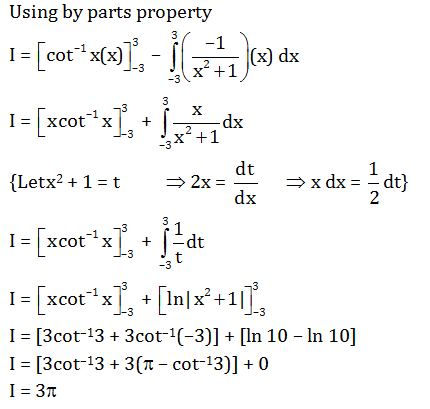Question 27:

$$\begin{array}{l}\int \frac{1}{\sqrt{x}+x\sqrt{x}}dx=\end{array}$$

a. 2 log (√x+1)+C
b. (½) tan-1√x+C
c. tan-1√x+C
d. 2 tan-1√x+C

Let I =

$$\begin{array}{l}\int \frac{1}{\sqrt{x}+x\sqrt{x}}dx\end{array}$$
$$\begin{array}{l}\int \frac{1}{\sqrt{x}(x+1)}dx\end{array}$$

Let √x = t

x = t2

dx = 2t dt

So I =

$$\begin{array}{l}\int \frac{1}{t(1+t^{2})}2t\: dt\end{array}$$

I =

$$\begin{array}{l}2\int \frac{1}{(1+t^{2})}\: dt\end{array}$$

= 2 tan-1t +C

= 2 tan-1√x+C

Question 28:

$$\begin{array}{l}\int \frac{2x-1}{(x-1)(x+2)(x-3)}dx=A\: log\left | x-1 \right |+B\: log\left | x+2 \right |+C\: log \left | x-3 \right |+K\end{array}$$
, then A, B, C are respectively:

a. -1/6, 1/3, -1/2
b. 1/6, 1/3, -1/5
c. 1/6, -1/3, 1/3
d. -1/6, -1/3, 1/2

Let I =

$$\begin{array}{l}\int \frac{2x-1}{(x-1)(x+2)(x-3)}dx\end{array}$$

Now,

(2x-1)/(x-1)(x+2)(x-3) = (A/(x-1) )+(B/(x+2))+(C/(x-3))

2x-1 = A(x+2)(x-3)+B(x-1)(x-3)+C(x -1)(x+2)

Putting x = 3, we get

6 -1 = C(3-1)(3+2)

C = ½

Putting x = 1, we get

2-1 = A(1+2)(1-3)

A = -1/6

Putting x = -2, we get

-4-1 = B(-2-1)(-2-3)

B = -1/3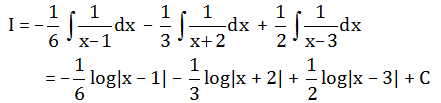A.B,C are -1/6, -1/3, 1/2.

Question 29:

$$\begin{array}{l}\int_{0}^{2}\left [ x^{2} \right ]dx=\end{array}$$

a. 5-√2-√3
b. 5+√2-√3
c. 5-√2+√3
d. -5-√2-√3

Let I =

$$\begin{array}{l}\int_{0}^{2}\left [ x^{2} \right ]dx\end{array}$$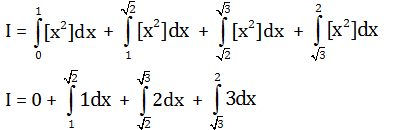I = (√2-1)+2(√3-√2)+3(2-√3)

= √2-1+2√3-2√2+6-3√3

= 5-√2-√3

Question 30:

$$\begin{array}{l}\int_{0}^{1}\sqrt{\frac{1+x}{1-x}}dx=\end{array}$$

a. (π/2)-1
b. (π/2)+1
c. π/2
d. 1/2

Let I =

$$\begin{array}{l}\int_{0}^{1}\sqrt{\frac{1+x}{1-x}}dx\end{array}$$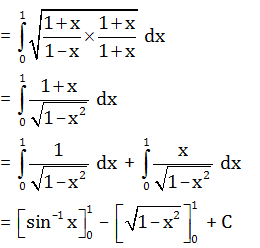= (π/2)-0-(0-1)

= (π/2)+1

Question 31: If α and β are the roots of the equation x2+x+1 = 0 then α22 is:>

a. 1
b. (-1+i√3)/2
c. (-1-i√3)/2
d. -1

Given equation is x2+x+1 = 0

α+β = -1

αβ = 1

Now, α22 = (α+β)2-2αβ

= (-1)2-2(1)

= 1-2

= -1

Question 32: The number of 4-digit numbers without repetition that can be formed using the digits 1,2,3,4,5,6,7 in which each number has two odd digits and two even digits is

a. 432
b. 436
c. 450
d. 454

Given digits are 1, 2, 3, 4, 5, 6, 7

Two even digits can be selected in 3C2

Two odd digits can be selected in 4C2 ways

These selected 4 digits can be arranged in 4! ways

The total number of ways = 4C2 ×3C2 ×4!

= 6×3×24

= 432

Question 33: The number of terms in the expansion of (x2+y2)25-(x2-y2)25 after simplification is

a. 0
b. 13
c. 26
d. 50

(x2+y2)25-(x2-y2)25

(a+b)n-(a-b)n if n is odd

On simplification we get (n+1)/2 terms

i.e. (25+1)/2 = 26/2

= 13

Question 34: The third term of a G.P. is 9. The product of its first five terms i

a. 35
b. 39
c. 310
d. 312

The third term of G.P. is 9

a.2 = 9

Now, the product of five terms = T1T2T3T4T5

= (a)(ar)(ar2)(ar3)(ar4)

= a5r10

= (ar2)5

= (9)5

= 310

Question 35: A line cuts off equal intercepts on the co-ordinate axes. The angle made by this line with the positive direction of X-axis is

a. 450
b. 900
c. 1200
d. 1350

We know that equation of a line in intercept form is

(x/a)+(y/b) = 1

Here, both intercept are equal

So (x/a)+(y/a) = 1

y = –x+a

Now comparing it with

y = mx + c

We get m = -1 = slope

We know that

Slope = tan θ

tan θ = -1

θ = 1350

Question 36:.

$$\begin{array}{l}\int x^{3}\sin 3xdx=\end{array}$$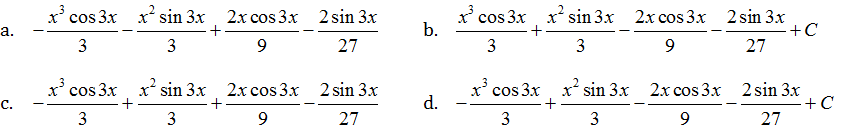Let I =

$$\begin{array}{l}\int x^{3}\sin 3xdx\end{array}$$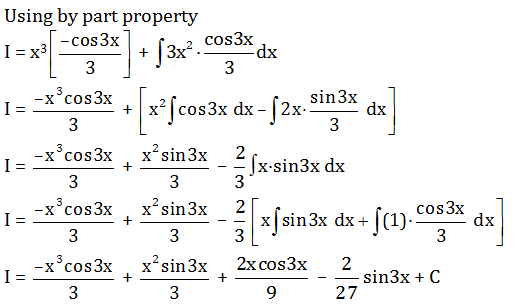Question 37: The area of the region above X-axis included between the parabola y2= x and the circle x2+y2 = 2x in square units is

a. (π/4)-(3/2)
b. (3/2)-( π/4)
c. (2/3)-( π/4)
d. (π/4)-(2/3)

y2 = x …(1)

x2+ y2 = 2x…(2)

(x-1)2 +(y-0)2 = 1

Equation (2) is a circle with centre (1, 0) and radius 1.

Solving eq.(1) and eq.(2)

(x-1)2 + x = 1

x2-x = 0

x(x-1) = 0

x = 0, 1

We get the points of intersection (0, 0) and (1, 1)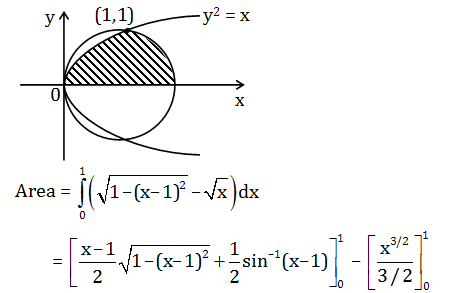= [0+ π/4]-(2/3)

= (-2/3)+( π/4)

Question 38: The area of the region bounded by Y-axis, y = cos x and y = sin x; 0 ≤xπ/2 is

a. √2-1 Sq. units
b. √2 Sq. units
c. √2+1 sq. units
d. 2-√2 Sq. units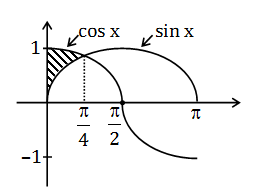Required area =

$$\begin{array}{l}\int_{0}^{\frac{\pi }{4}}(\cos x-\sin x)dx\end{array}$$

=

$$\begin{array}{l}[\cos x-\sin x]_{0}^{\frac{\pi }{4}}\end{array}$$

= (1/√2)+(1/√2)-1)

= (√2-1) Sq. units

Question 39: The integrating factor of the differential equation (2x+3y2)dy = y dx (y > 0) is

a. e1/y
b. -1/y2
c. 1/x
d. 1/y2

(2x+3y2)dy = y dx

(2x+3y2)/y = dx/dy

(dx/dy)-(2/y)x = 3y

I.F =

$$\begin{array}{l}e^{\int \frac{-2}{y}dy}\end{array}$$

= e-2 log y

= y-2

= 1/y2

Question 40: The equation of the curve passing through the point (1,1) such that the slope of the tangent at any point (x,y) is equal to the product of its coordinates is

a. 2 log x = y2-1
b. 2 log y = x2+1
c. 2 log y = x2-1
d. 2 log x = y2+1

(dy/dx) = xy

(1/y)dy = x dx

Integrate

log y = (x2/2)+C ..(i)

Equation (i) passing through (1,2)

log(1) = (12/2)+C

C = -1/2

Put in (i)

log y = (x2/2)-(1/2)

2 log y = x2-1

Question 41: The eccentricity of the ellipse 9x2+25y2 = 225 is

a. 4/5
b. 3/5
c. ¾
d. 9/16

9x2+25y2 = 225

(x2/52)+(y2/32) = 1

Eccentricity (e) = √(a2-b2)/a

= √(25-9)/5

= 4/5

Question 42:

$$\begin{array}{l}\sum_{r=1}^{n}(2r-1)=x\end{array}$$
then
$$\begin{array}{l}\lim_{n \to \infty }\left [ \frac{1^{3}}{x^{2}} +\frac{2^{3}}{x^{2}}+\frac{3^{3}}{x^{2}}+…+\frac{n^{3}}{x^{2}}\right ] =\end{array}$$

a. ½
b. ¼
c. 1
d. 4

$$\begin{array}{l}\sum_{r=1}^{n}(2r-1)=x\end{array}$$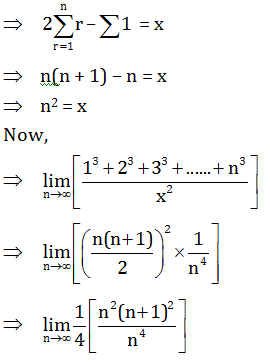= (1/4)×1

= 1/4

Question 43: The negation of the statement ”All continuous functions are differentiable.”

a. All continuous functions are not differentiable
b. Some continuous functions are differentiable.
c. Some continuous functions are not differentiable.
d. All differentiable functions are continuous.

Always all differentiable functions are continuous. But all continuous functions are not differentiable. So, negation of the given statement is some continuous functions are not differentiable. Ex: |x|

Question 44: Mean and standard deviation of 100 items are 50 and 4 respectively. The sum of all squares of the items is

a. 251600
b. 256100
c. 266000
d. 261600

Here n = 100

A = 50

σ = 4

Now A = Σx/n

Σx = An

Σx = 100×50

= 5000

Again from the formula,

σ2+A2 = Σx2/n

Σx2 = n(σ2+A2)

= 100(16+2500)

= 251600

Question 45: Two letters are chosen from the letters of the word ‘EQUATIONS’. The probability that one is vowel and the other is consonant is

a. 8/9
b. 4/9
c. 3/9
d. 5/9

Vowel: E, A, U, I, O ⇒ P(v) = 5C1

Consonant: Q, T, N, S ⇒ P(c) = 4C1

Probability = 5C1 × 4C1/9C2

= 5×4/36

= 20/36

= 5/9

Question 46: The constant term in the expansion of

$$\begin{array}{l}\begin{vmatrix} 3x+1 & 2x-1 &x+2 \\ 5x-1 & 3x+2 & x+1\\ 7x-2 & 3x+1 & 4x-1 \end{vmatrix}\end{array}$$
is:

a. 0
b. 2
c. -10
d. 6

$$\begin{array}{l}\Delta =\begin{vmatrix} 3x+1 & 2x-1 &x+2 \\ 5x-1 & 3x+2 & x+1\\ 7x-2 & 3x+1 & 4x-1 \end{vmatrix}\end{array}$$

Put x = 0

$$\begin{array}{l}\Delta =\begin{vmatrix} 1 & -1 &2 \\ -1 & 2 & 1\\ -2& 1 & -1 \end{vmatrix}\end{array}$$
$$\begin{array}{l}\Delta\end{array}$$
= 1(-3)+1(3)+2(-1+4)

= -3+3+6

= 6

Question 47: If [x] represents the greatest integer function and f(x) = x-[x]-cos x then f’(π/2) =

a. 0
b. 1
c. 2
d. does not exist

f(x) = x-[x]-cos x

since x = [x]+{x}

f(x) = {x}-cos x

f’(x) = 1+sin x

f’(π/2) = 1+ sin (π/2)

= 1+1

= 2

Question 48: If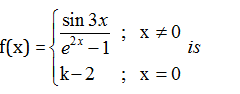continuous at x=0, then k=

a. 3/2
b. 9/5
c. 1/2
d. 2/3

Bonus

*G indicates one grace mark

Question 49: If f(x) = sin-1[(2x+1 )/(1+4x)], then f’(0) =

a. 2 log 2
b. log 2
c. (2 log 2)/5
d. (4 log 2)/5

f(x) = sin-1[(2x+1 )/(1+4x)]

= sin-1[(2×2x)/(1+(2x)2)]

= 2 tan-1(2x)

Differentiating w.r.t.x

f’(x) = (2/(1+4x))(2x log 2)

f’(0) = (2/(1+1)) log 2

f’(0) = log 2

Question 50: If x = a sec2 θ, y = a tan2 θ then d2y/dx2 =

a. 2a
b. 1
c. 0
d. 4

x = a sec2 θ

y = a tan2 θ

y = a(sec2 θ-1)

y = a(x-1)

dy/dx = a

d2y/dx2 = 0

Question 51: The inverse of the matrix

$$\begin{array}{l}\begin{bmatrix} 2 &5 &0 \\ 0 & 1 &1 \\ -1 & 0 & 3 \end{bmatrix}\end{array}$$
is:

a.

$$\begin{array}{l}\begin{bmatrix} 3 & -1 & 1\\ -15& 6 &-5 \\ 5 & -2 & 2 \end{bmatrix}\end{array}$$

b.
$$\begin{array}{l}\begin{bmatrix} 3 & -5 & 5\\ -1& -6 &-2 \\ 1 & -5 & 2 \end{bmatrix}\end{array}$$

c.
$$\begin{array}{l}\begin{bmatrix} 3 & -15 & 5\\ -1 &6 & -2\\ 1& -5 & 2 \end{bmatrix}\end{array}$$

d.
$$\begin{array}{l}\begin{bmatrix} 3 & -15 & 5\\ -1 &6 & -2\\ 1& -5 & -2 \end{bmatrix}\end{array}$$

Let A =

$$\begin{array}{l}\begin{bmatrix} 2 &5 &0 \\ 0 & 1 &1 \\ -1 & 0 & 3 \end{bmatrix}\end{array}$$
$$\begin{array}{l}\left | A \right |=2(3-0)-5(0+1)+0\end{array}$$

= 1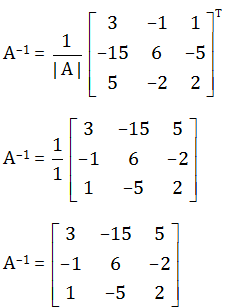Question 52: If P and Q are symmetric matrices of the same order then PQ-QP is

a. Identity matrix
b. Symmetric matrix
c. zero matrix
d. Skew symmetric matrix

Given P = P’ and Q = Q’

Now,

(PQ-QP)’ = (PQ)’-(QP)’

= Q’P’-P’Q’

= QP-PQ

= -[PQ-QP]

So (PQ-QP) is skew-symmetric.

Question 53: If 3A+4B’ =

$$\begin{array}{l}\begin{bmatrix} 7 & -10 & 17\\ 0 &6 & 31 \end{bmatrix}\end{array}$$
and 2B-3A’ =
$$\begin{array}{l}\begin{bmatrix} -1 & 18\\ 4 & 0\\ -5& -7 \end{bmatrix}\end{array}$$
then B =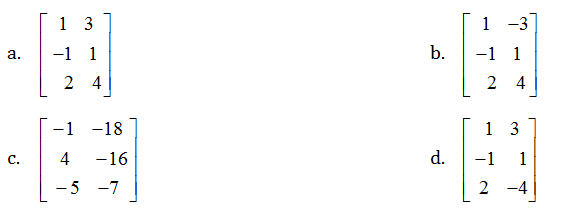3A+4B’ =

$$\begin{array}{l}\begin{bmatrix} 7 & -10 & 17\\ 0 &6 & 31 \end{bmatrix}\end{array}$$
..(1)

2B-3A’ =

$$\begin{array}{l}\begin{bmatrix} -1 & 18\\ 4 & 0\\ -5& -7 \end{bmatrix}\end{array}$$
..(2)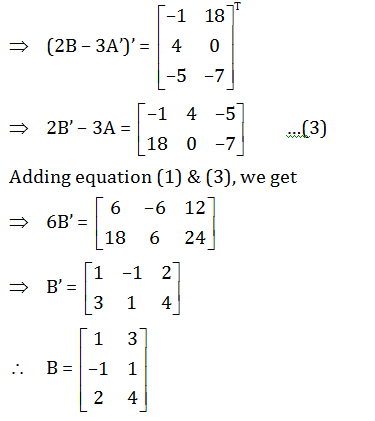Question 54: If A =

$$\begin{array}{l}\begin{bmatrix} 1 & 3\\ 4 & 2 \end{bmatrix}\end{array}$$
, B =
$$\begin{array}{l}\begin{bmatrix} 2 & -1\\ 1 & 2 \end{bmatrix}\end{array}$$
, then |ABB’|=

a. 50
b. -250
c. 100
d. 250

A =

$$\begin{array}{l}\begin{bmatrix} 1 & 3\\ 4 & 2 \end{bmatrix}\end{array}$$

B =

$$\begin{array}{l}\begin{bmatrix} 2 & -1\\ 1 & 2 \end{bmatrix}\end{array}$$

B’ =

$$\begin{array}{l}\begin{bmatrix} 2 & 1\\ -1 & 2 \end{bmatrix}\end{array}$$

Now, |ABB’| = |A| |B| |B’|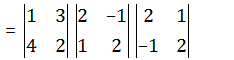= (2-12)(4+1)(4+1)

= -10×5×5

= -250

Question 55: If the value of a third-order determinant is 16, then the value of the determinant formed by replacing each of its elements by its cofactor is

a. 96
b. 48
c. 256
d. 16

Given that |A| = 16

Order of determinant A = 3

= 162

= 256

Question 56:

$$\begin{array}{l}f:R\rightarrow R\end{array}$$
and
$$\begin{array}{l}g:[0,\infty )\rightarrow R\end{array}$$
is defined by f(x) = x2 and g(x) = √x. Which one of the following is not true ?

a. gof (4) = 4
b. fog (-4) = 4
c. fog (2) = 2
d. gof (-2) = 2

f(x) = x2

g(x) = √x

Now,

fog(x) = f[g(x)]

= f[√x ]

= x

fog(2) = 2

fog(-4) = -4

And

gof(x) = g[f(x)]

gof(x) = g[x2]

gof(-2) = g(4)

= √4

= 2

Question 57: A = {x|x∈N, x ≤5}, B = {x|x∈Z, x2-5x+6 = 0}, then the number of onto functions from A to B is

a. 2
b. 23
c. 30
d. 32

A = {x|x∈N, x ≤5}

A = {1,2,3,4,5}

B = {x|x∈Z, x2-5x+6 = 0}

B = {2,3}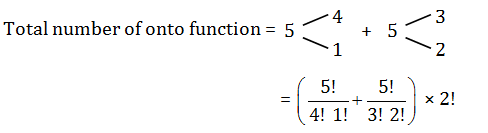= (5+10)×2

= 30

Question 58: On the set of positive rationals, a binary operation * is defined by a*b = 2ab/5 . If 2*x = 3-1, then x =

a. 1/6
b. 5/12
c. 2/5
d. 125/48

Given condition

a*b = 2ab/5

Now,

2*x = 3-1

2(2x)/5= 1/3

4x = 5/3

x = 5/12

Question 59: cos[2 sin-1 (3/4)+cos-1(3/4)] =

a. -3/4
b. 3/4
c. 3/5
d. does not exist

cos[2 sin-1 (3/4)+cos-1(3/4)] = cos [2 sin-1(3/4)+cos-1(3/4)]

= cos [sin-1(3/4)+ sin-1(3/4)+cos-1(3/4)]

= cos [(π/2)+sin-1(3/4)]

= -sin(sin-1(3/4)

= -3/4

Question 60: If a+ π/2 <2 tan-1x+3 cot-1x<b then ‘a’ and ‘b’ are respectively:

a. 0 and π
b. π/2 and 2π
c. 0 and 2π
d. -π/2 and π/2

We know that 0 < cot-1x < π

⇒ π+0<2 (π/2)+ cot-1x < π+ π

⇒ π<2(tan-1x+cot-1x)+cot-1x<2π

⇒ π<2tan-1x+3cot-1x<2π ..(1)

compare equation (1) to a+ π/2 <2 tan-1x+3 cot-1x<b

a = π/2

b = 2π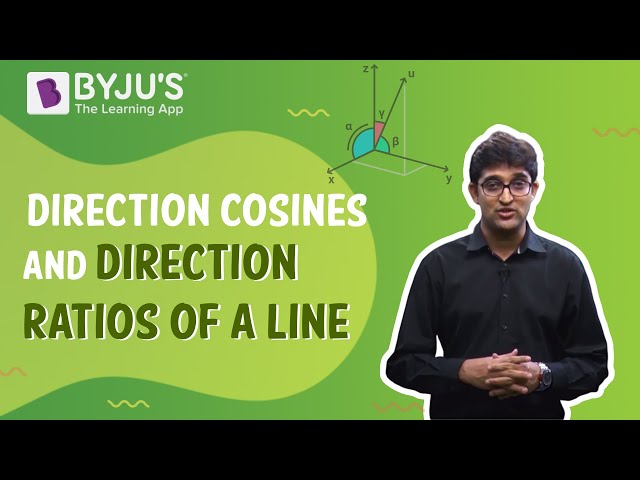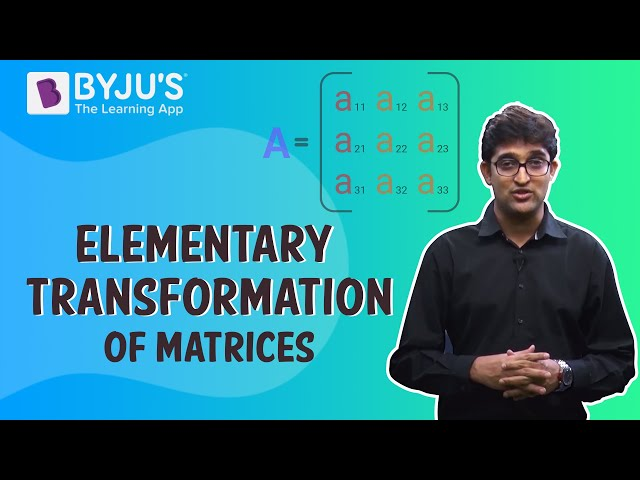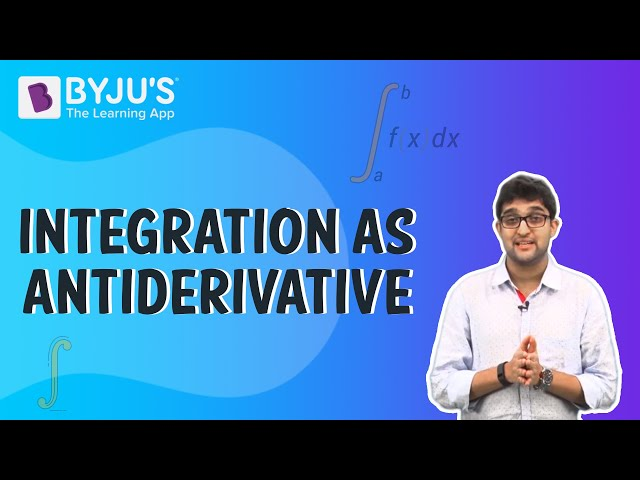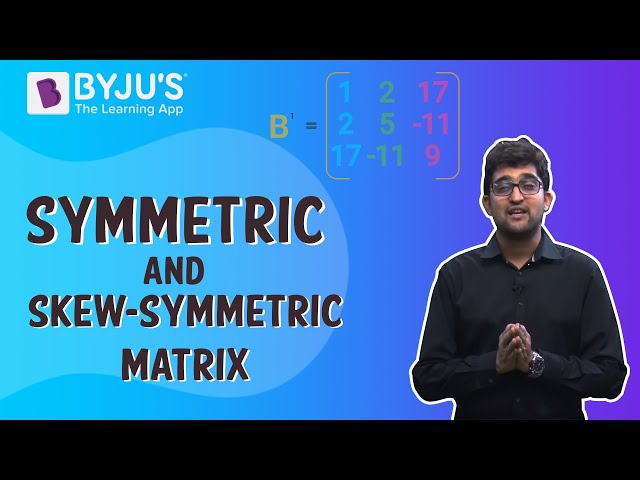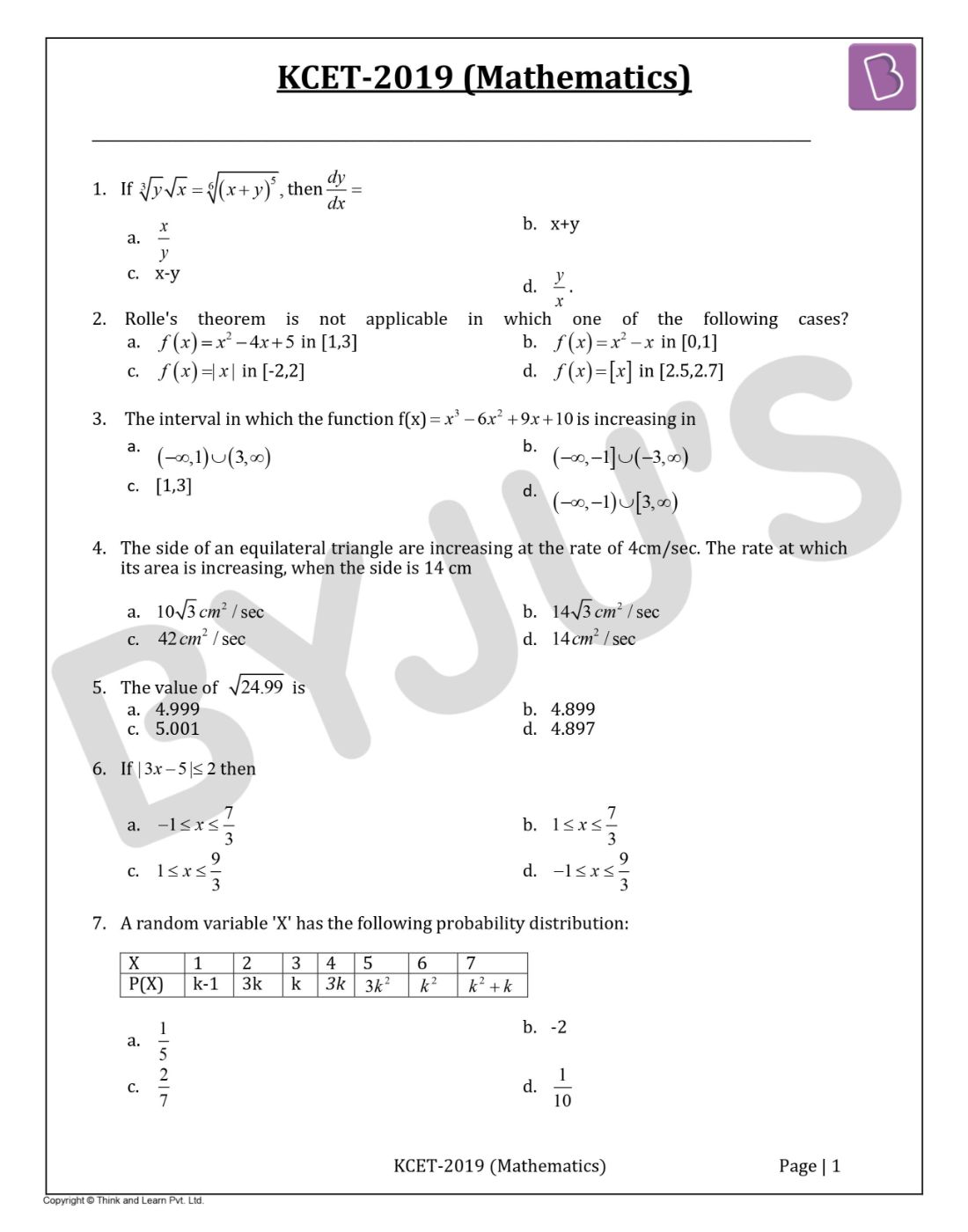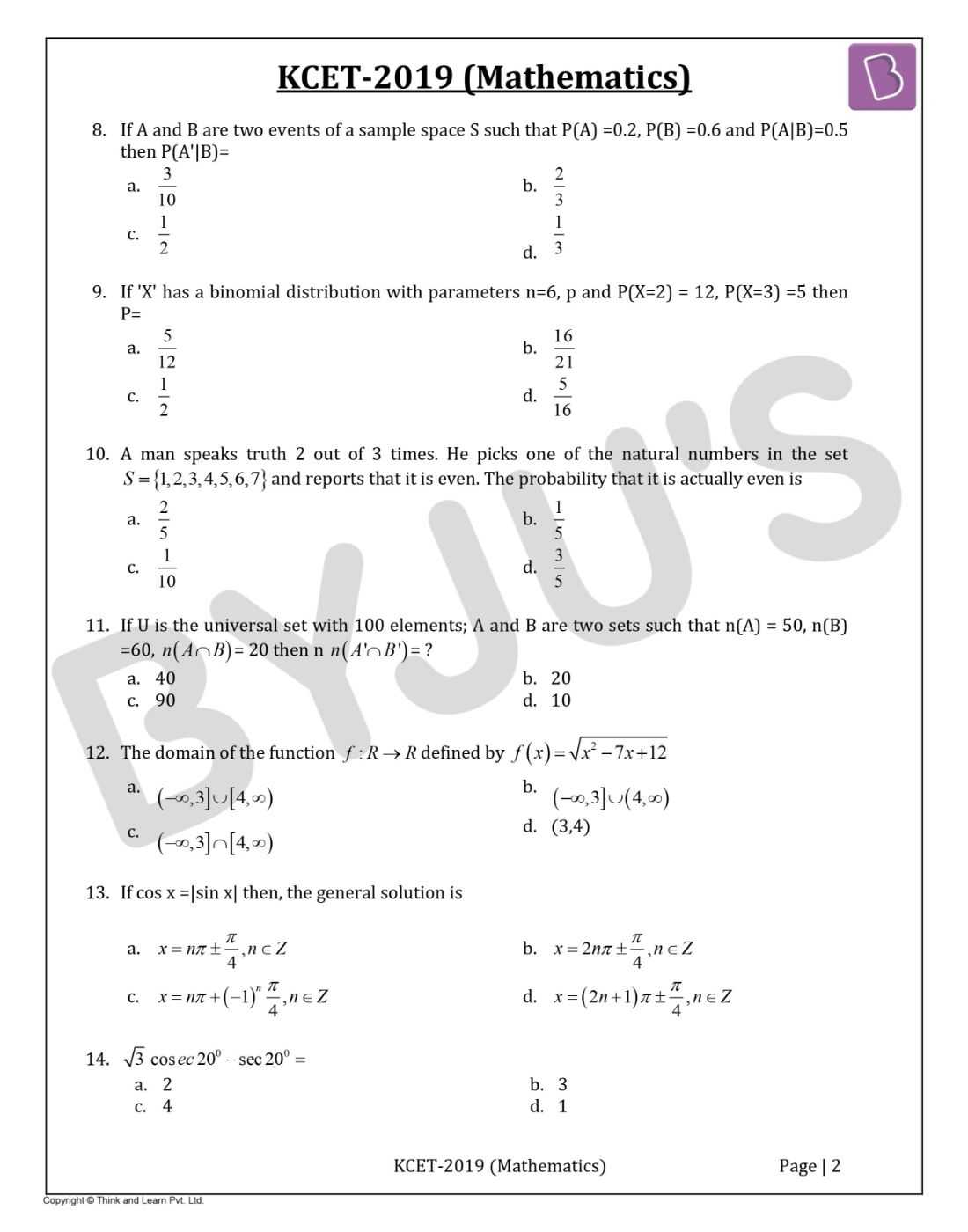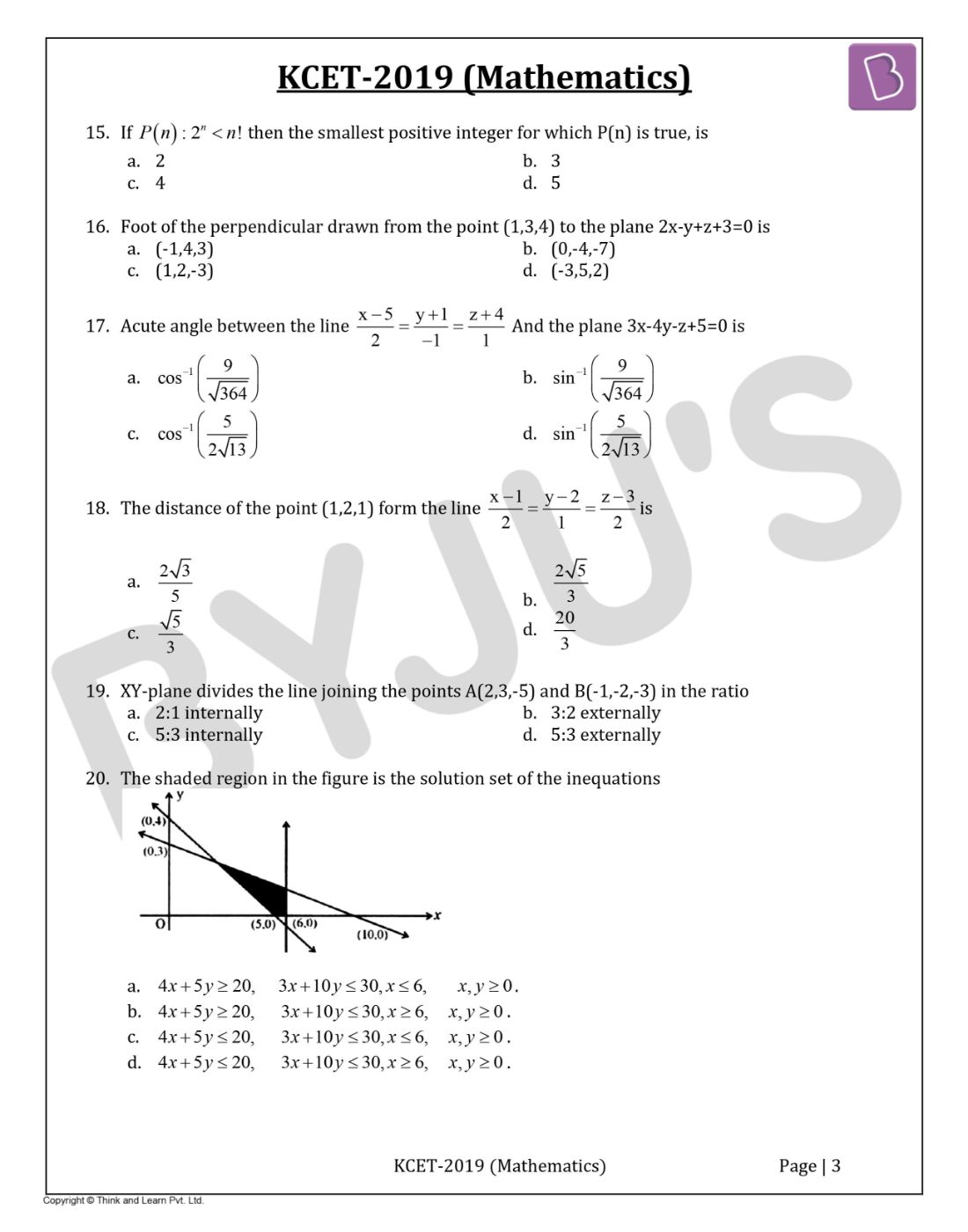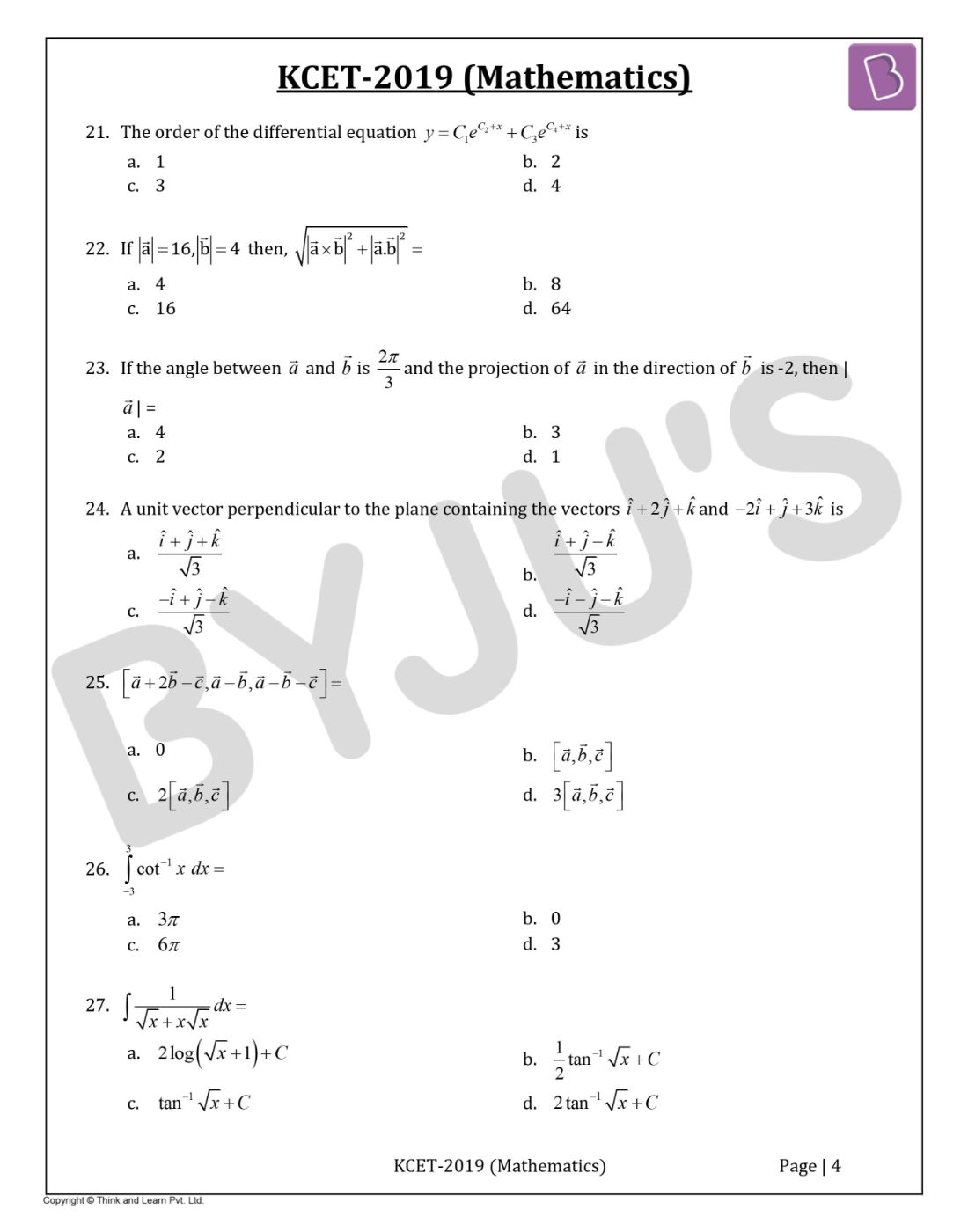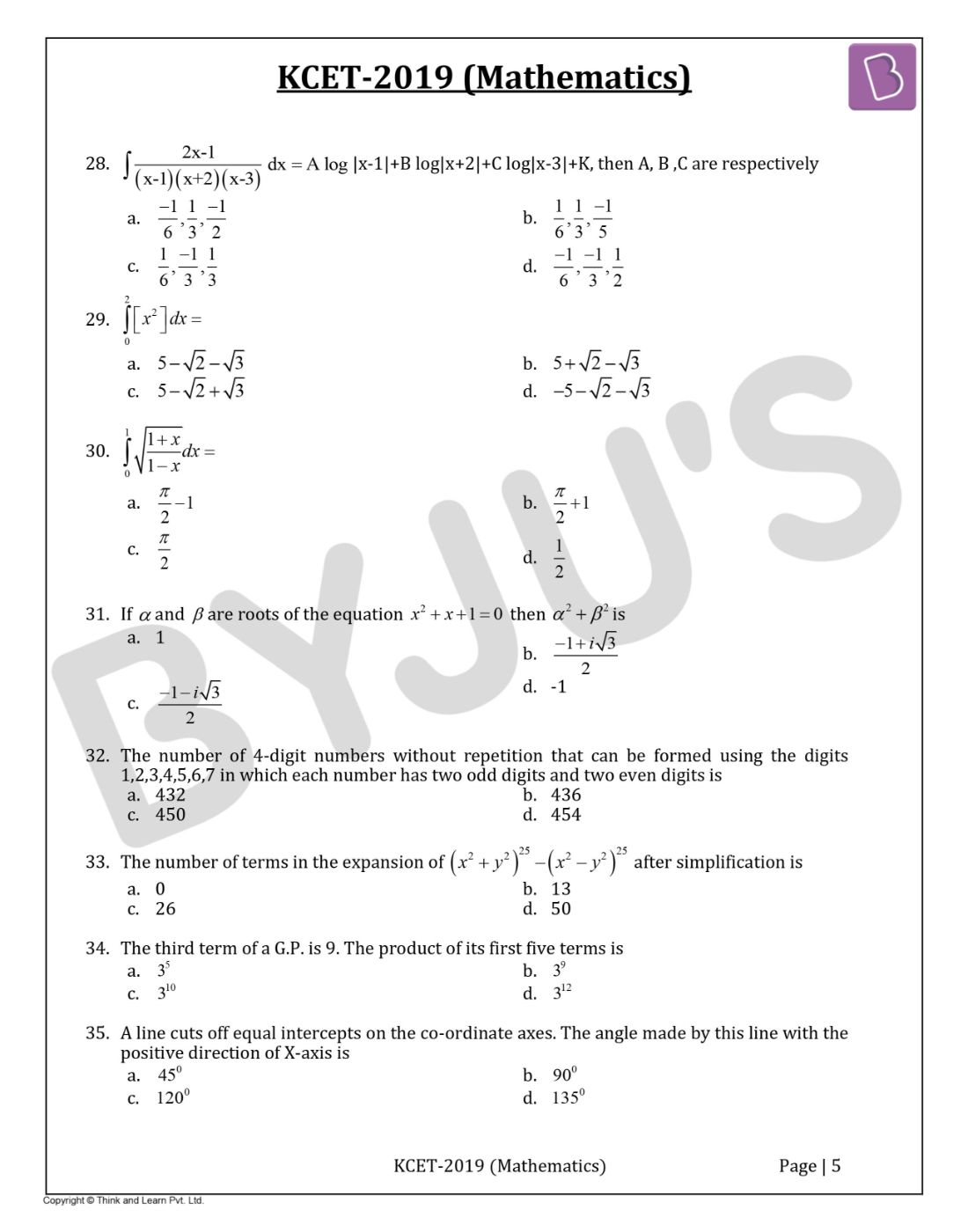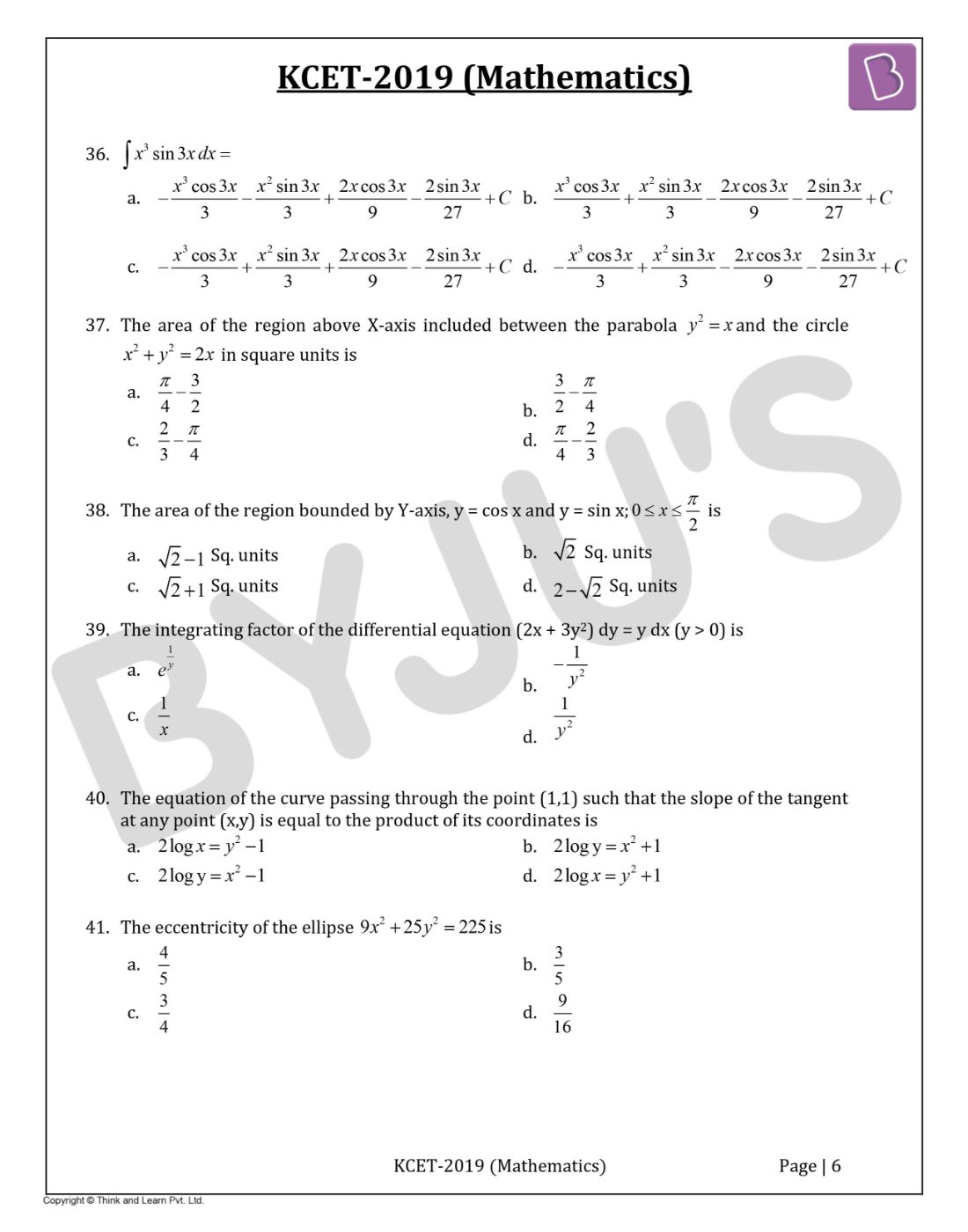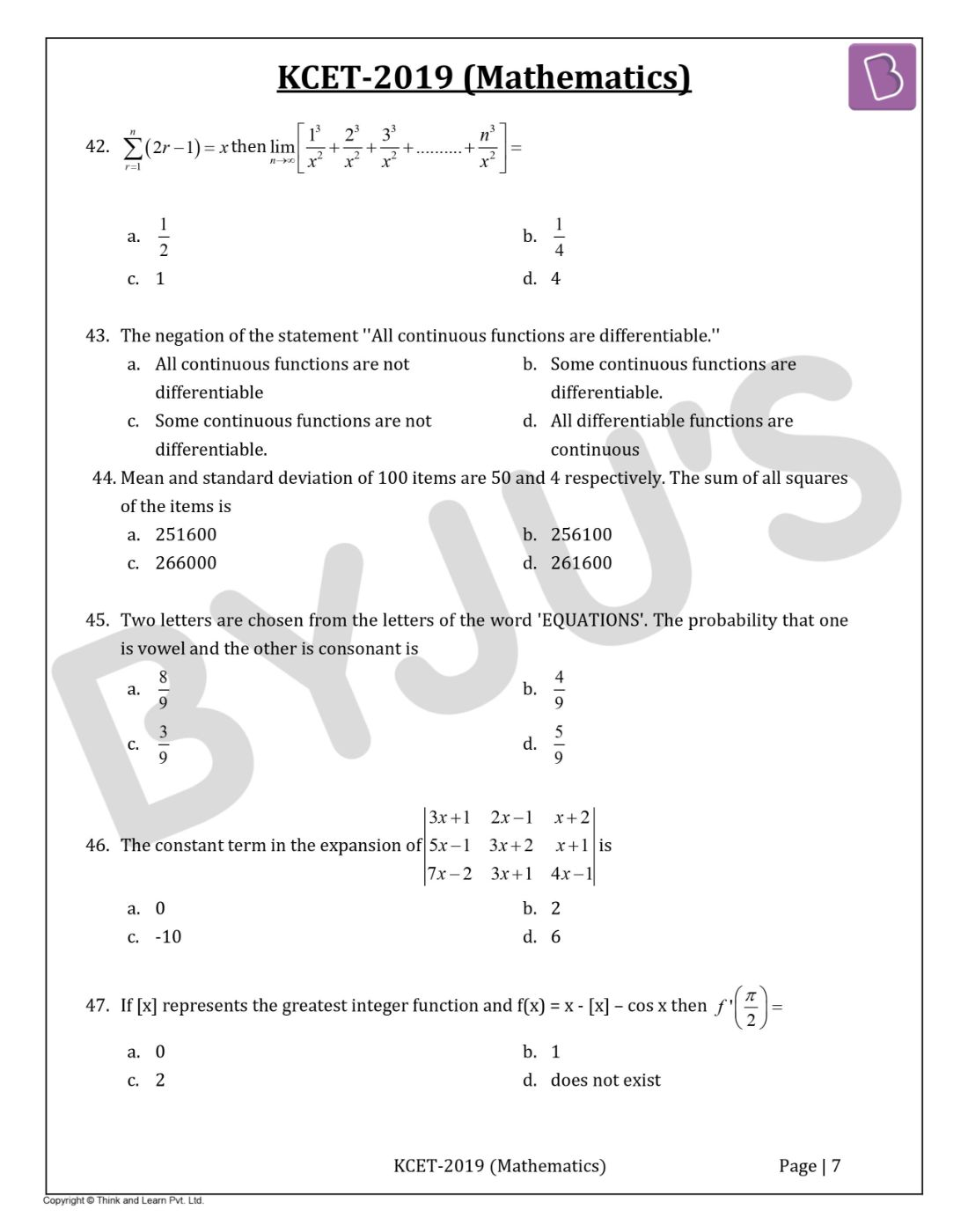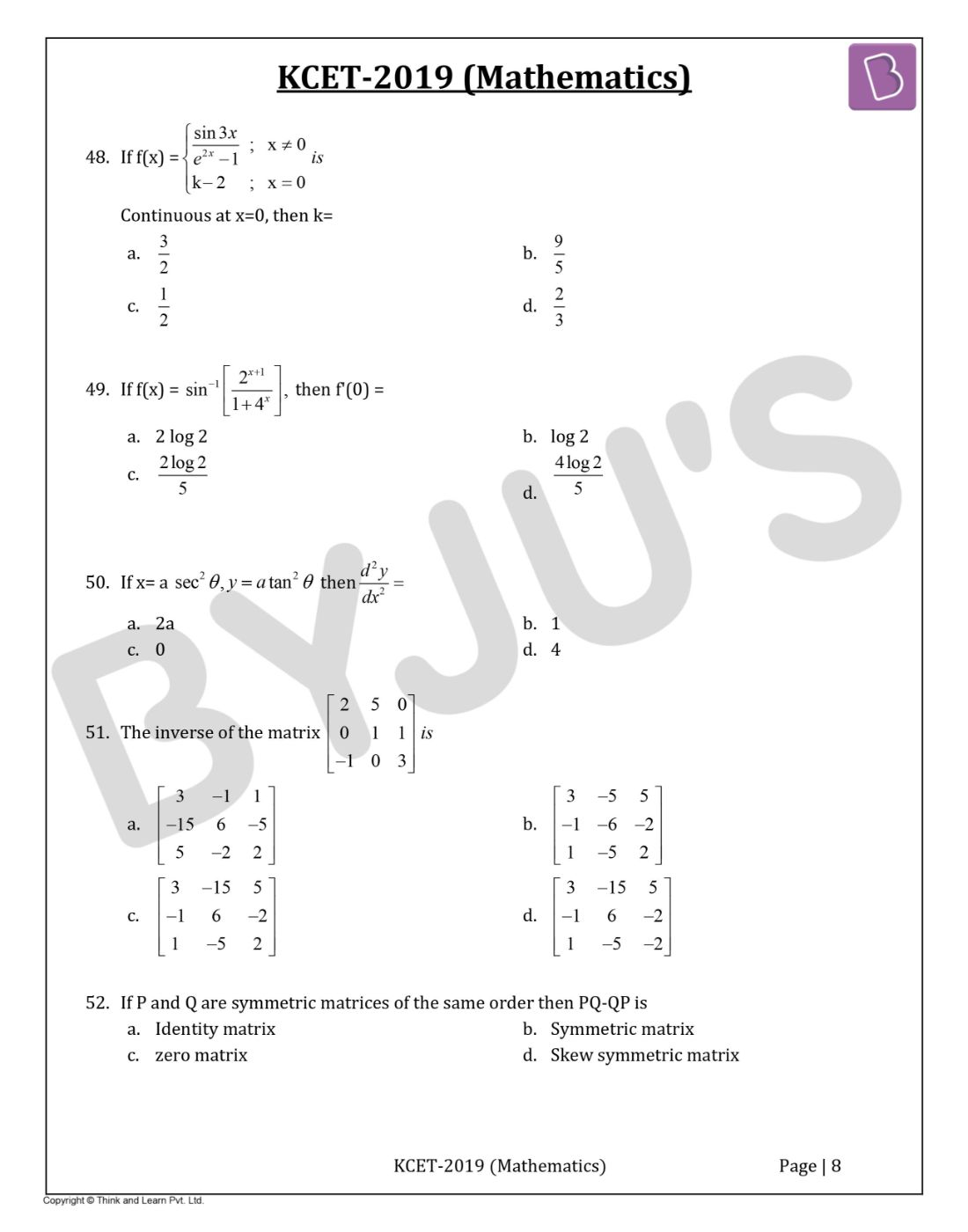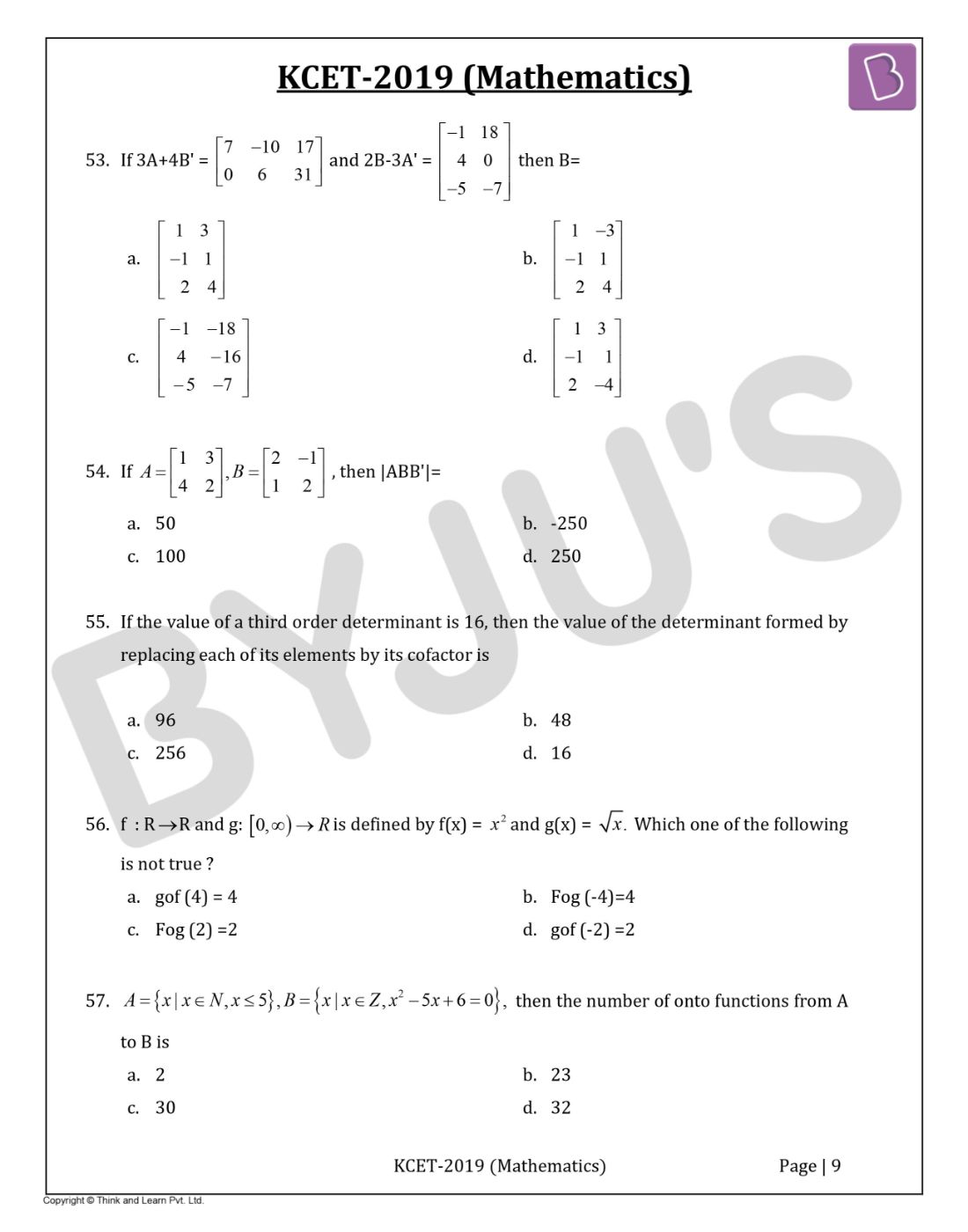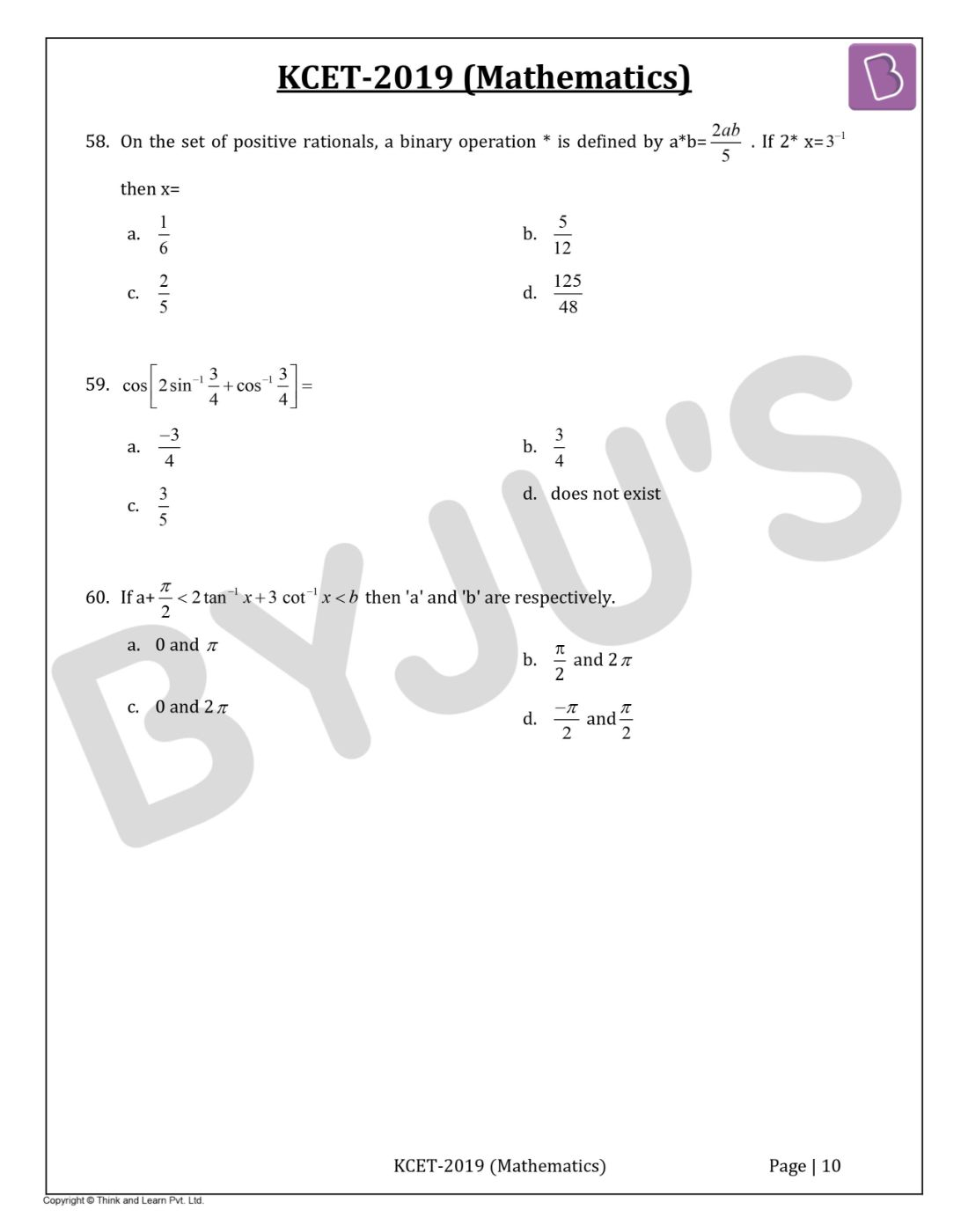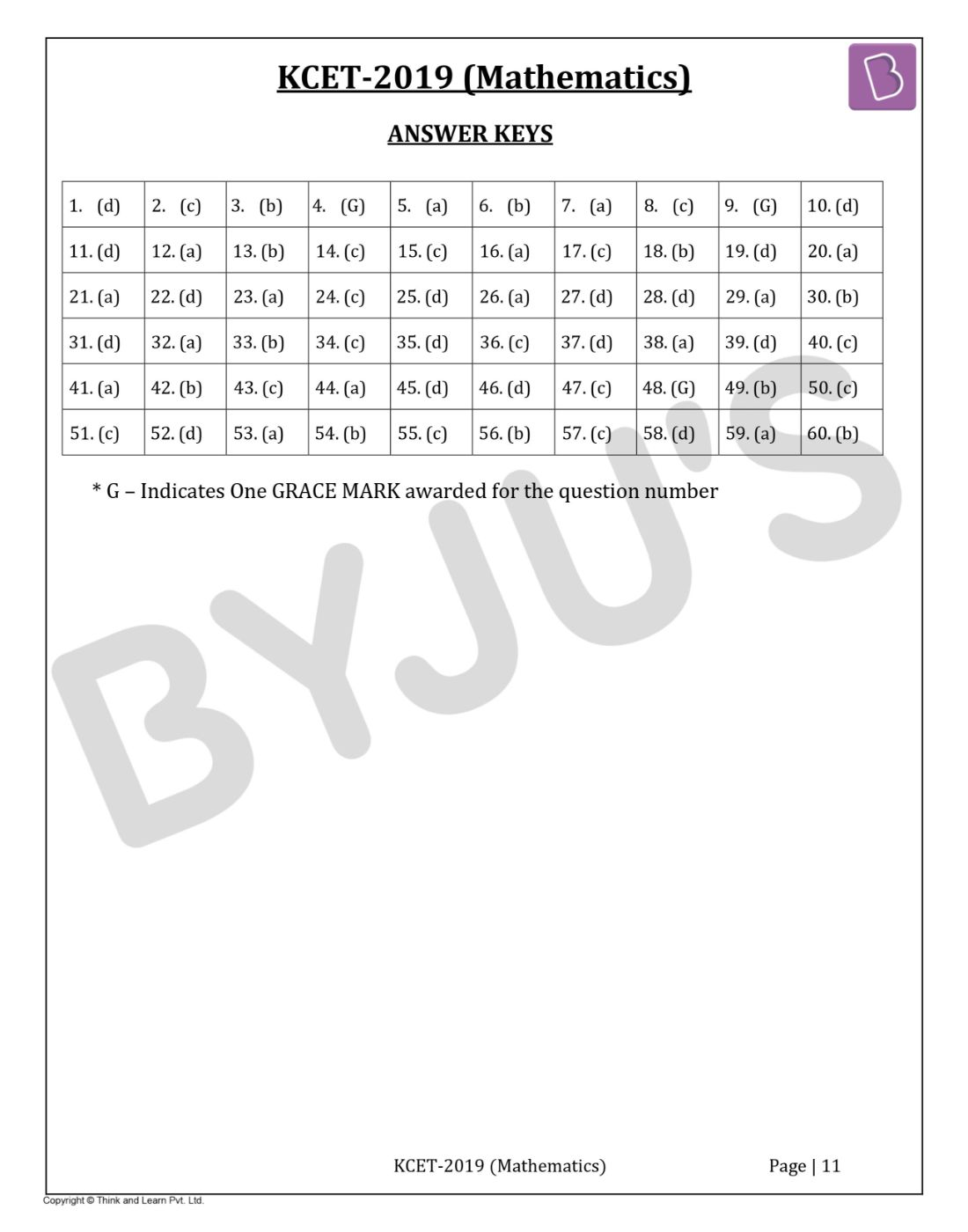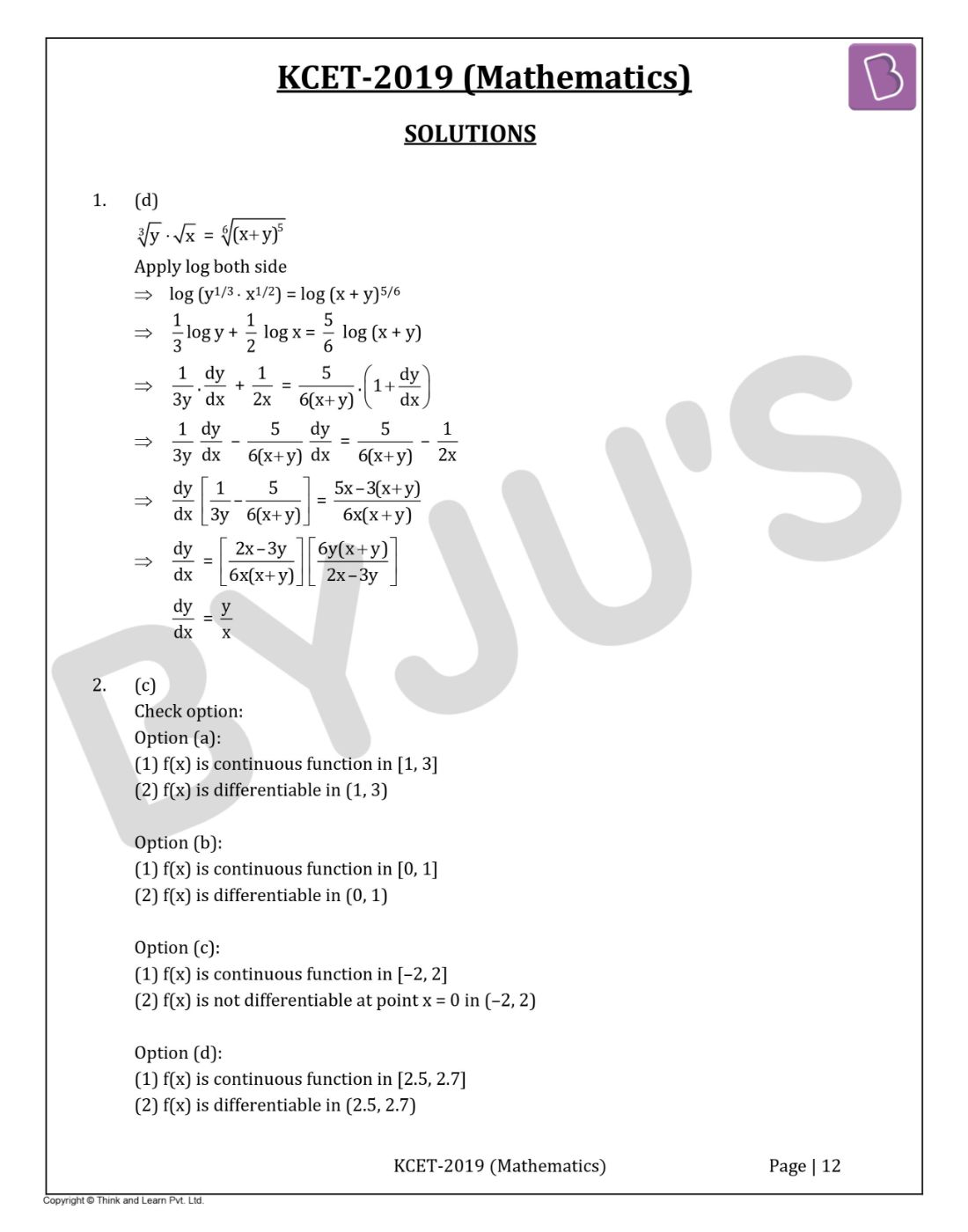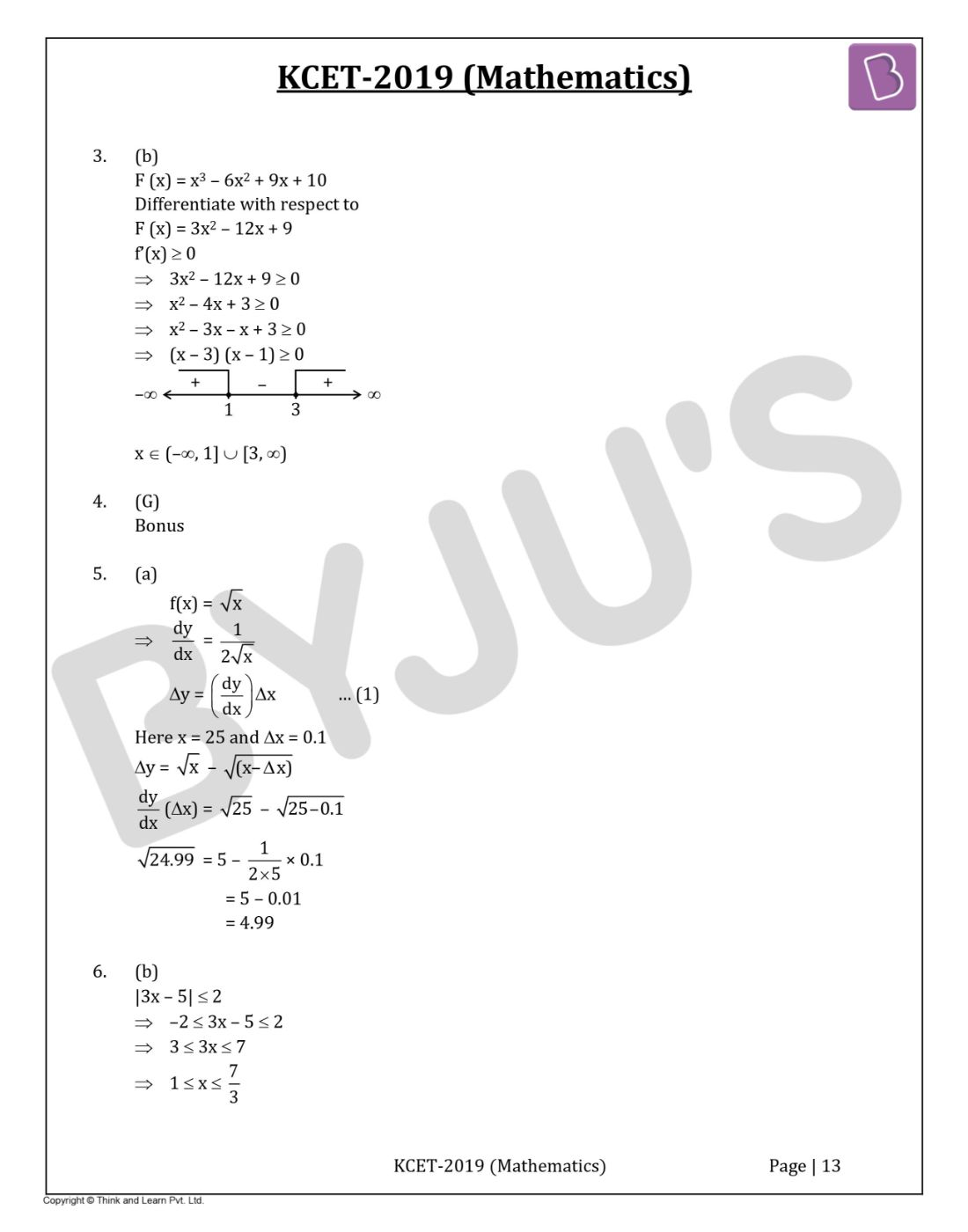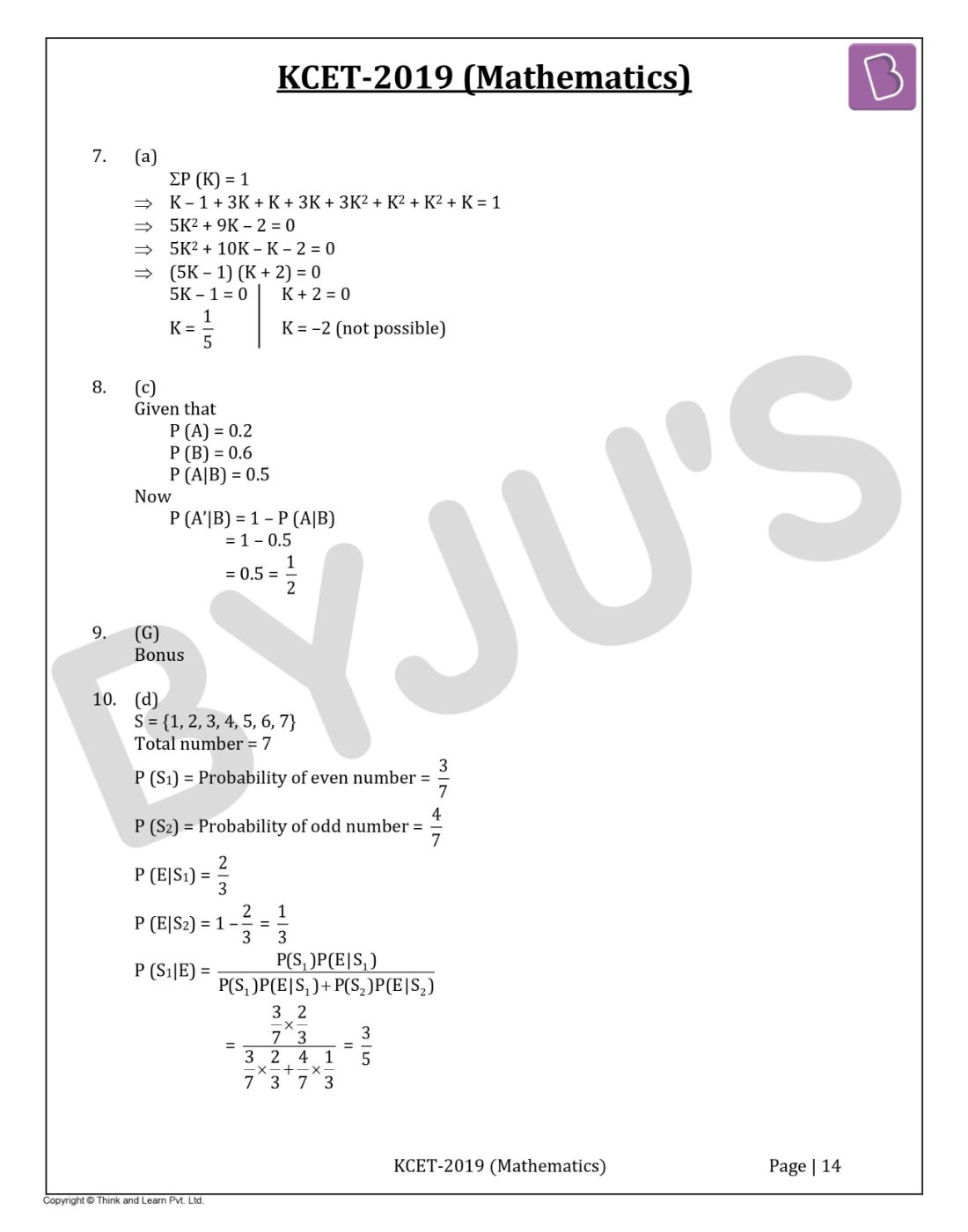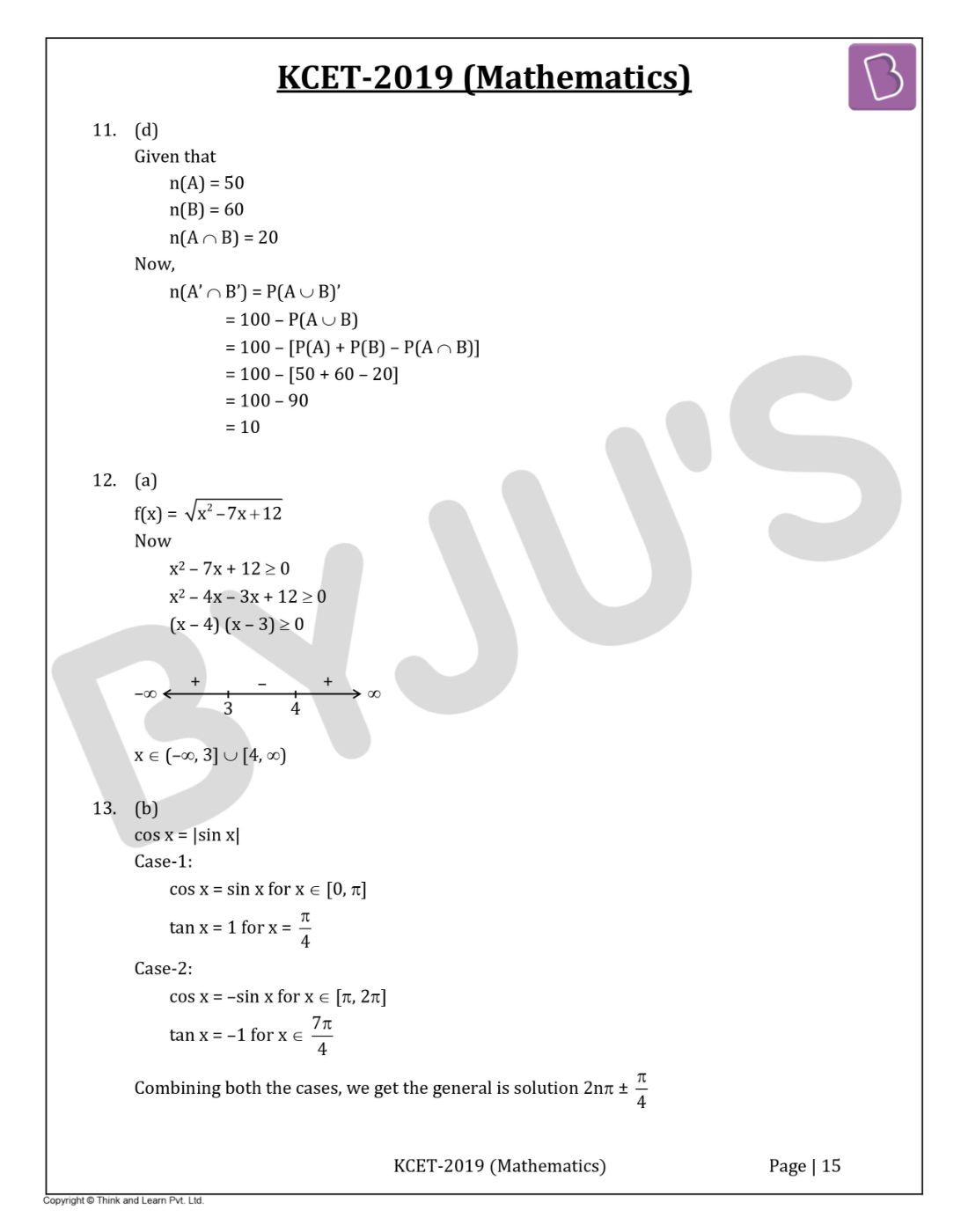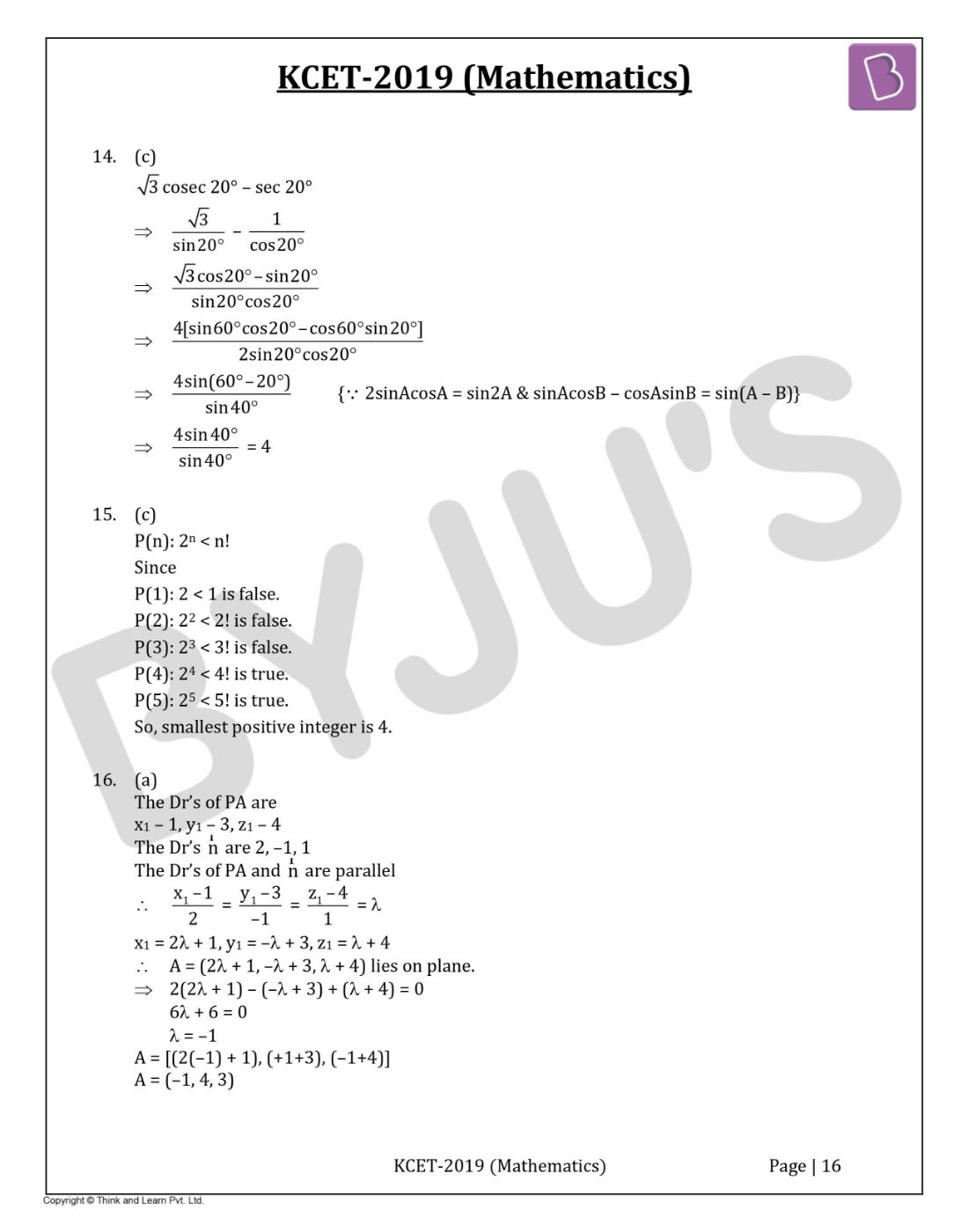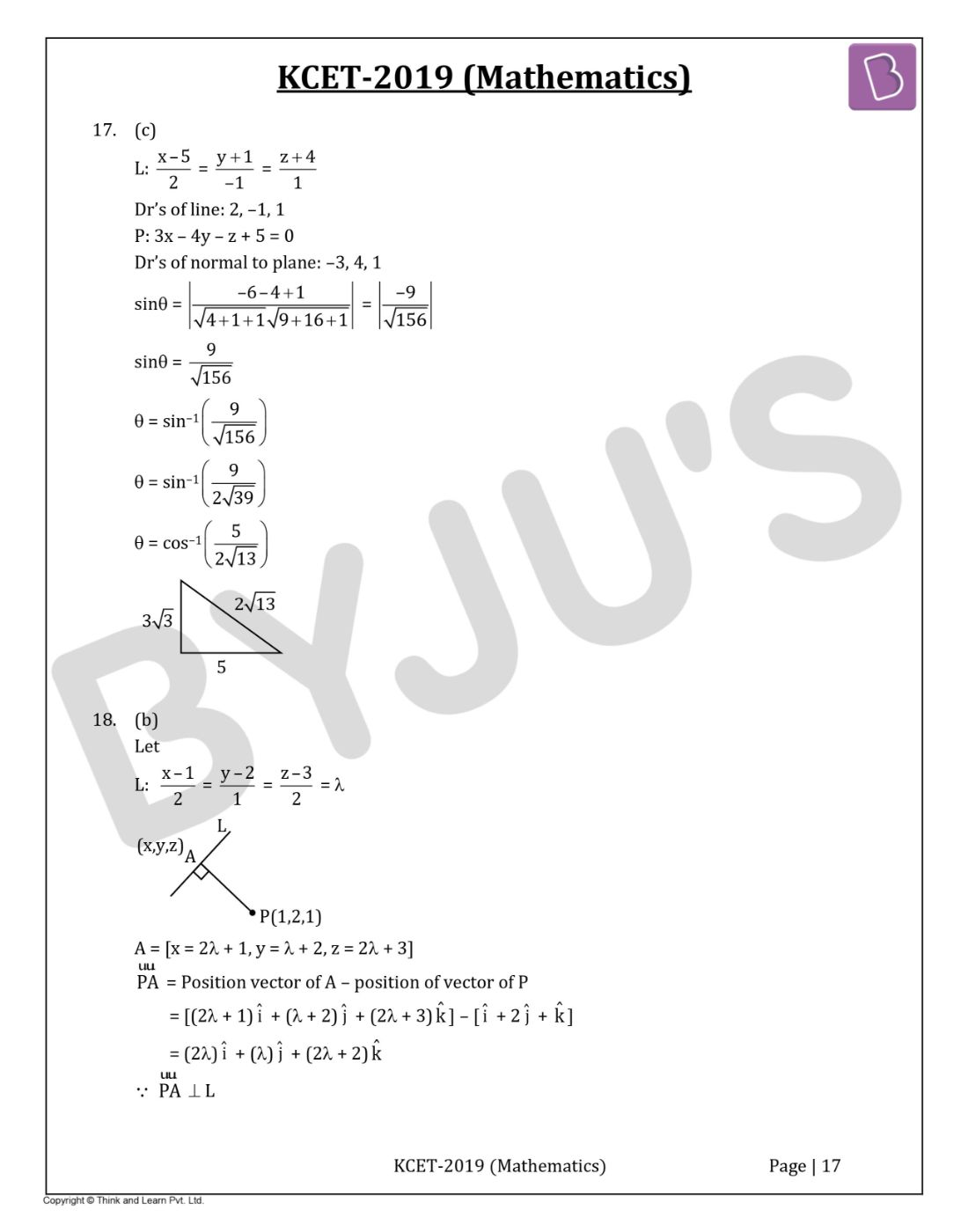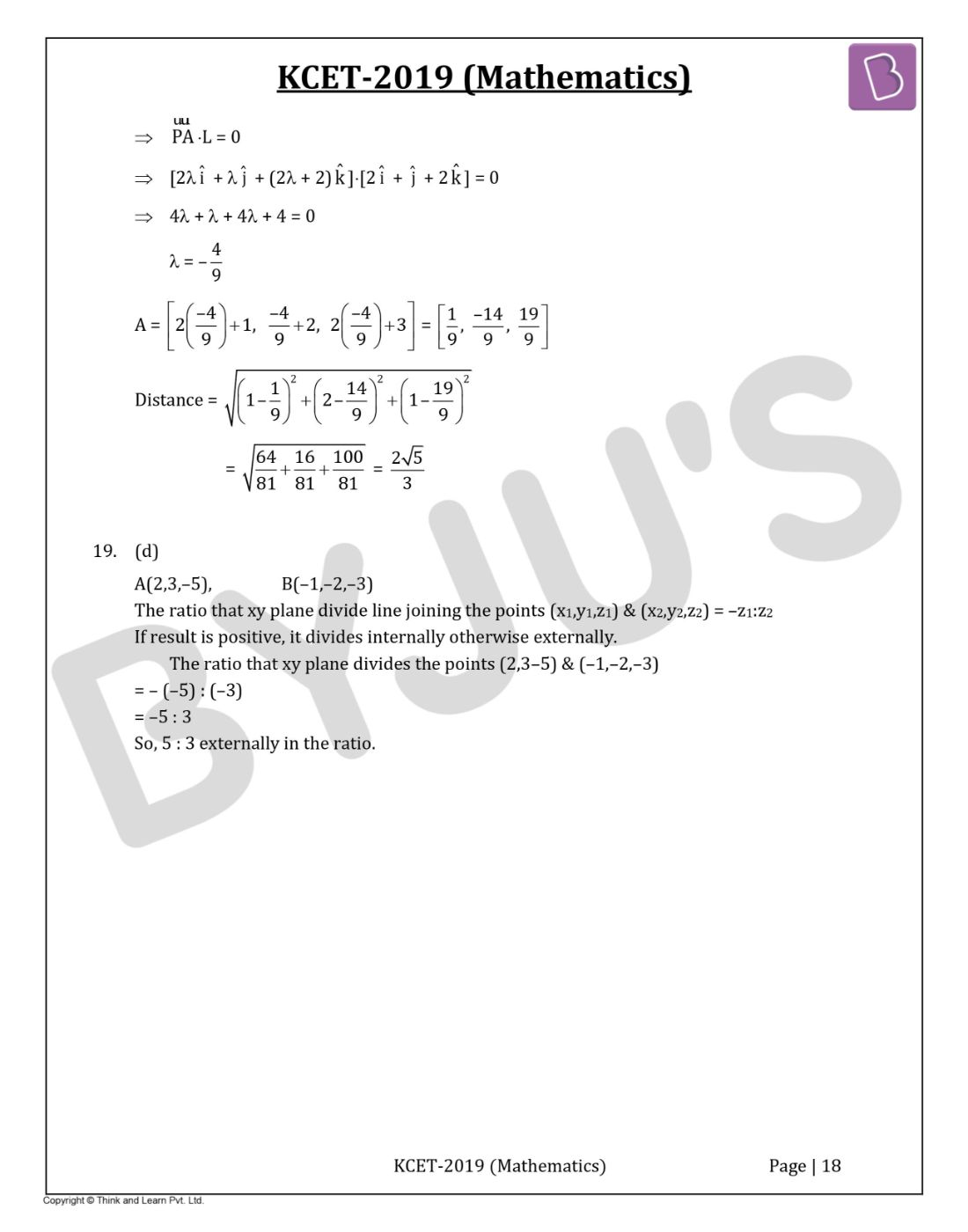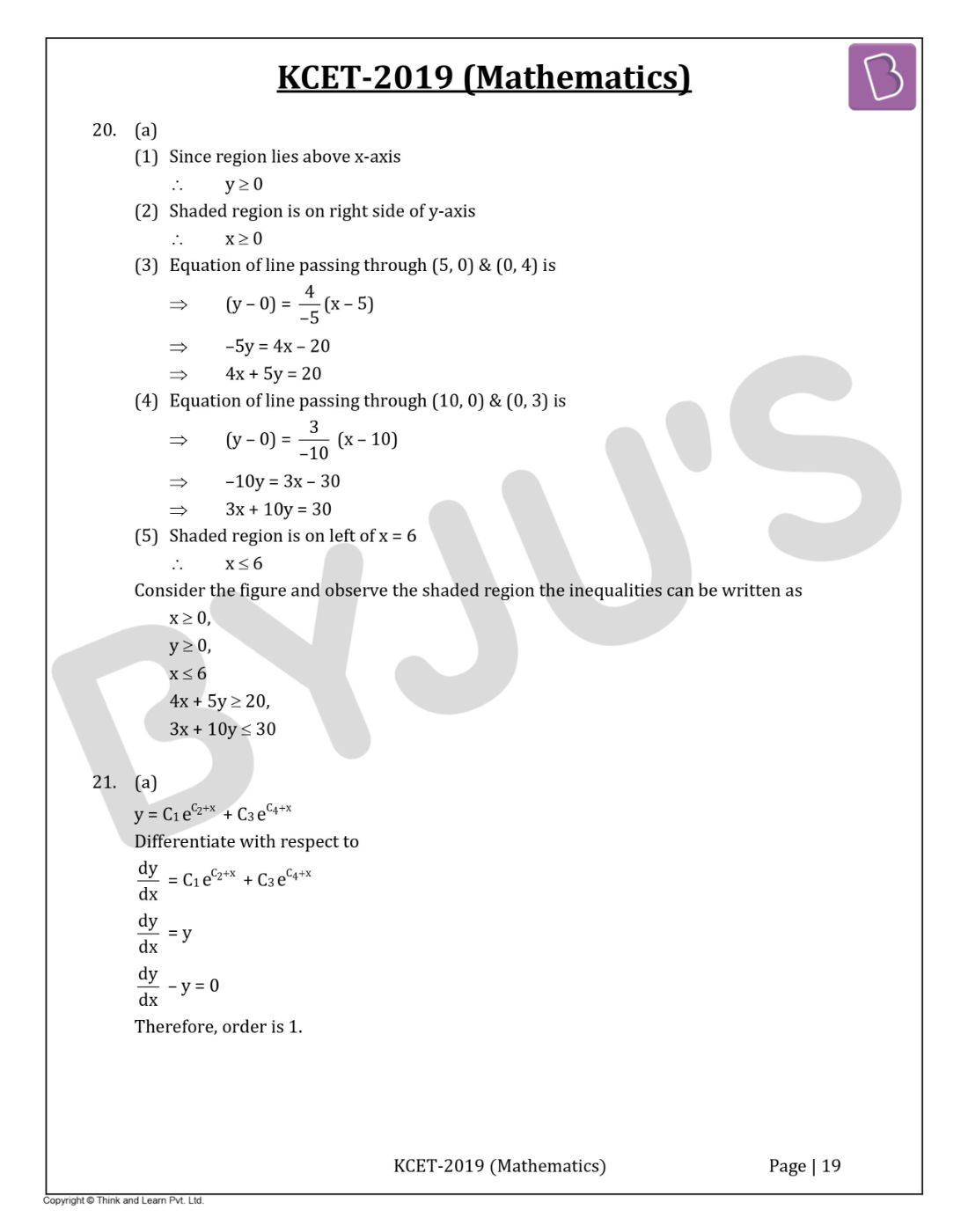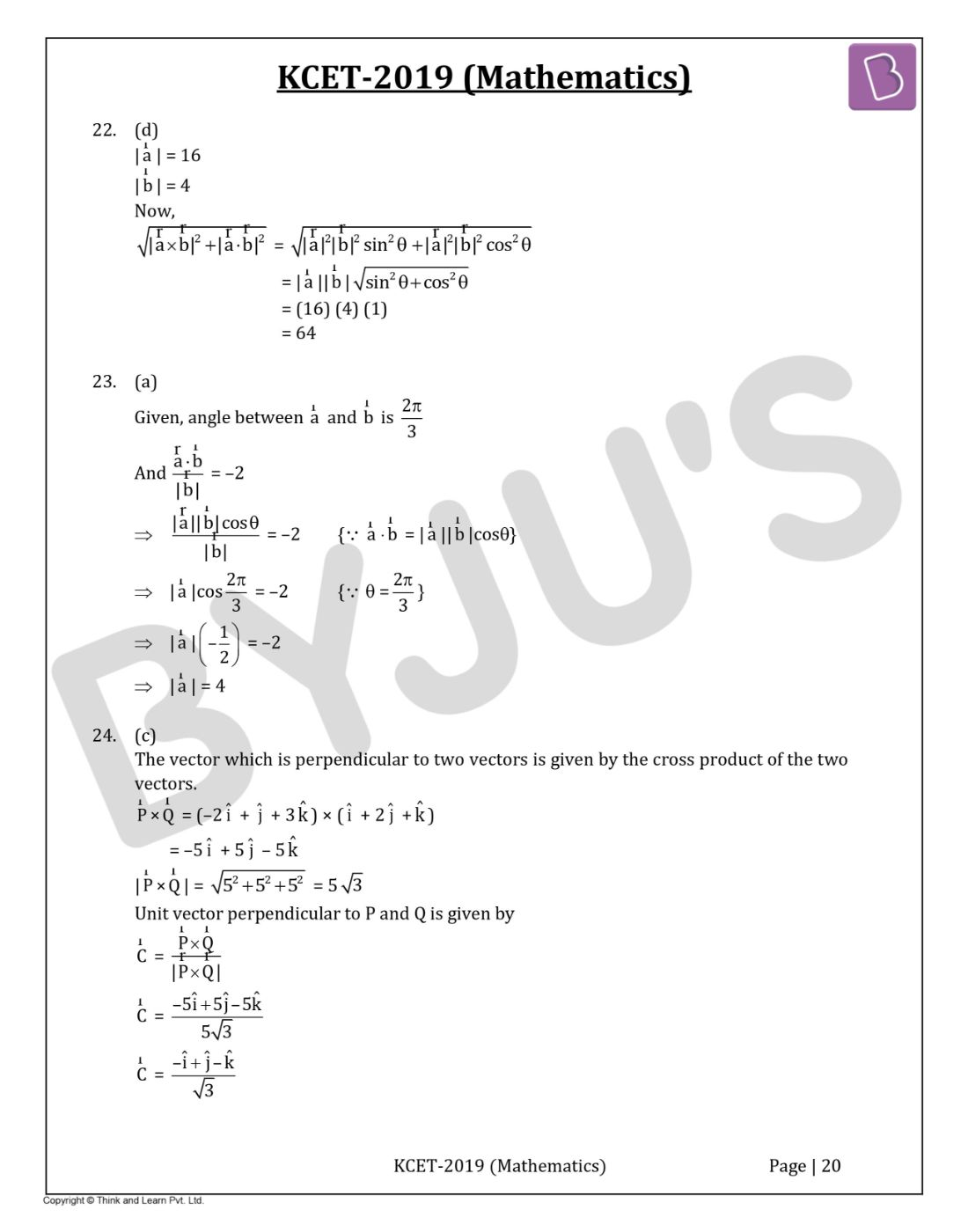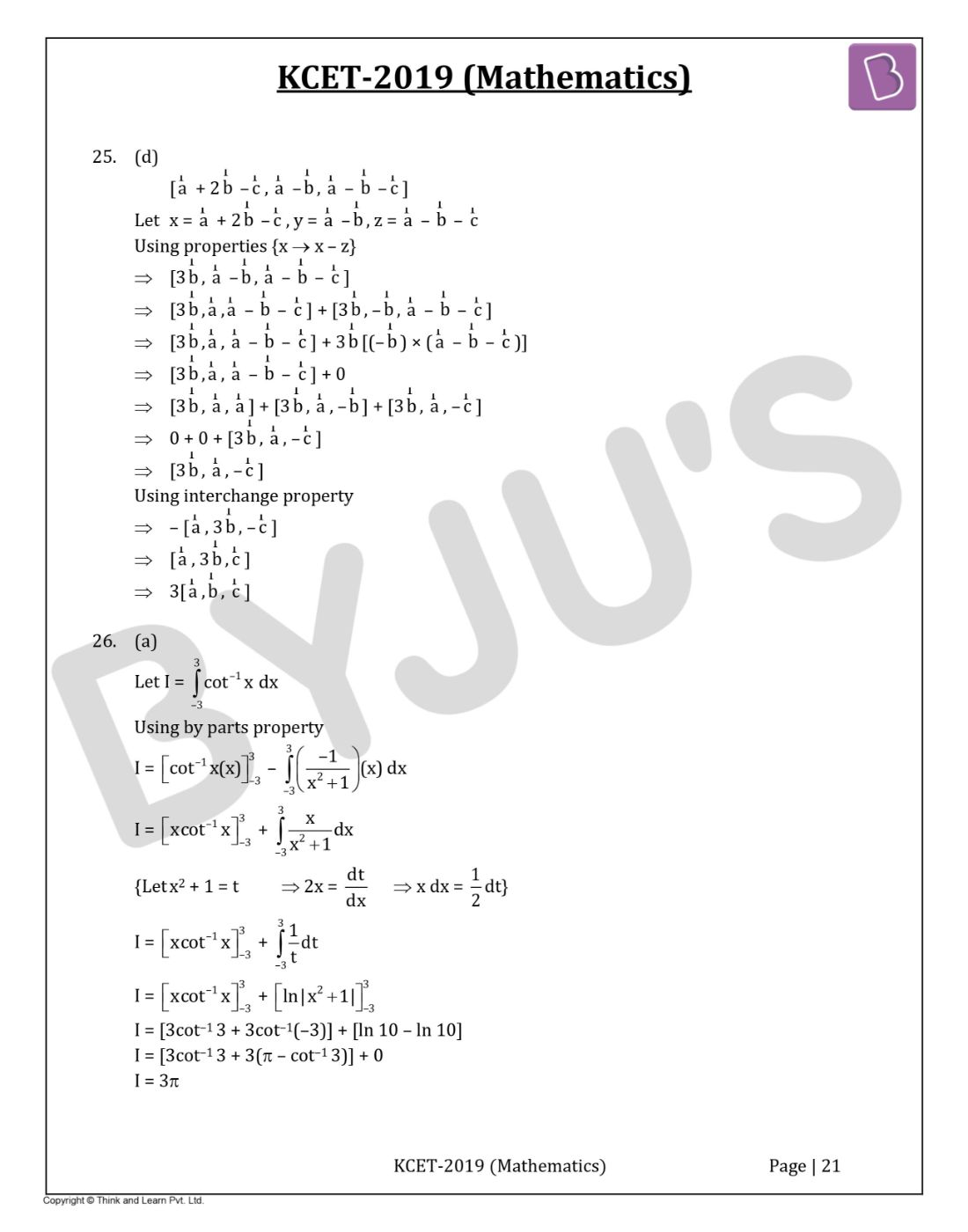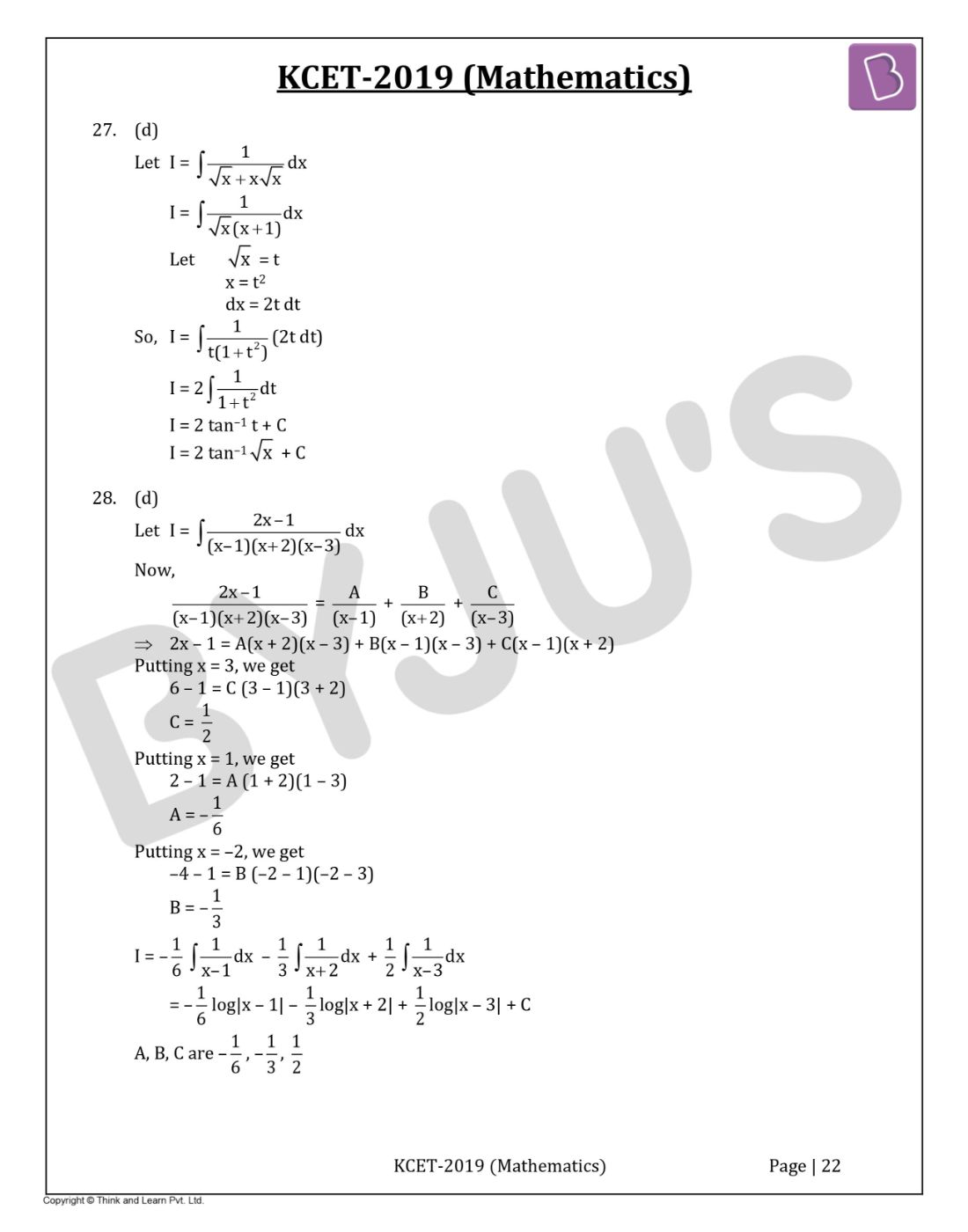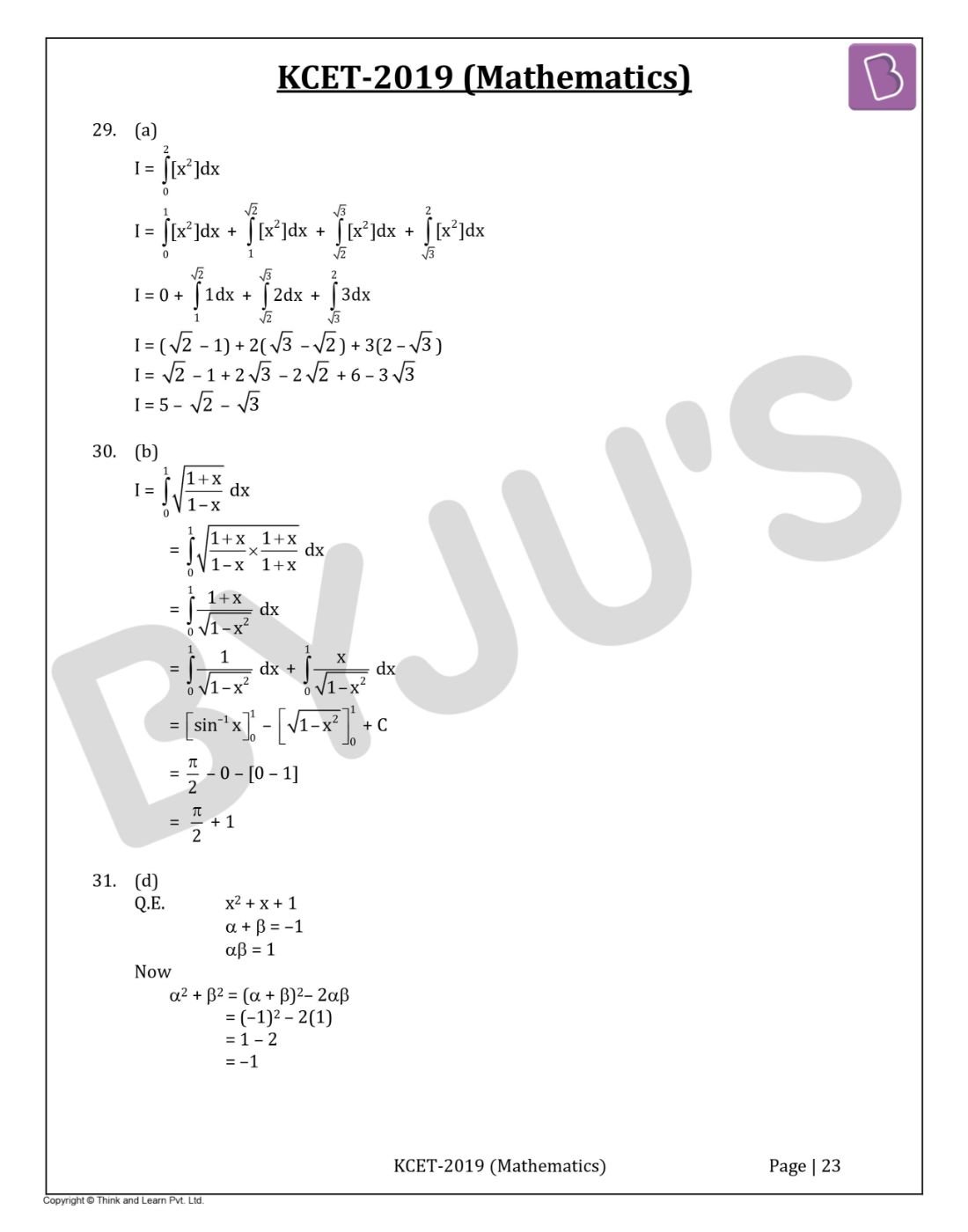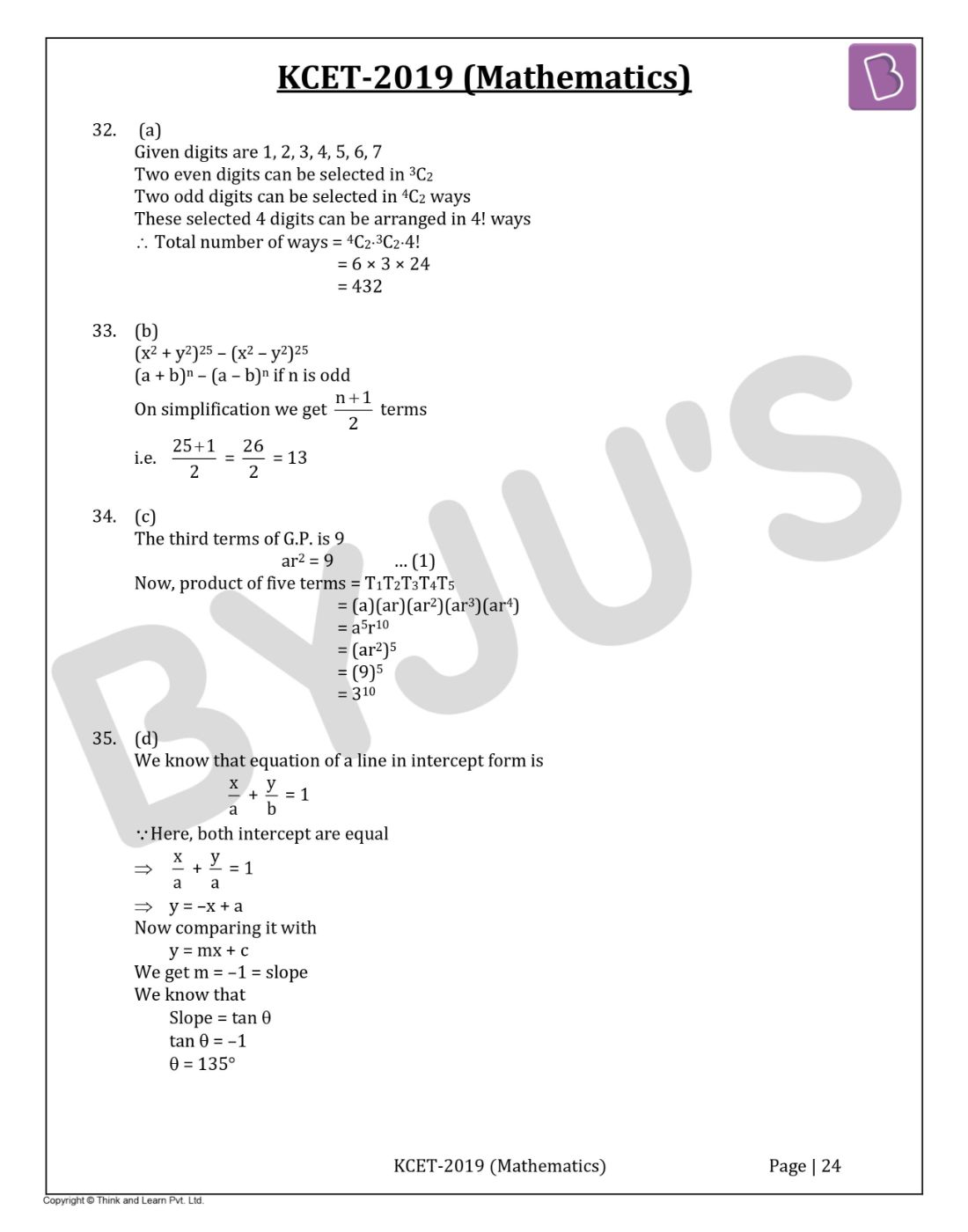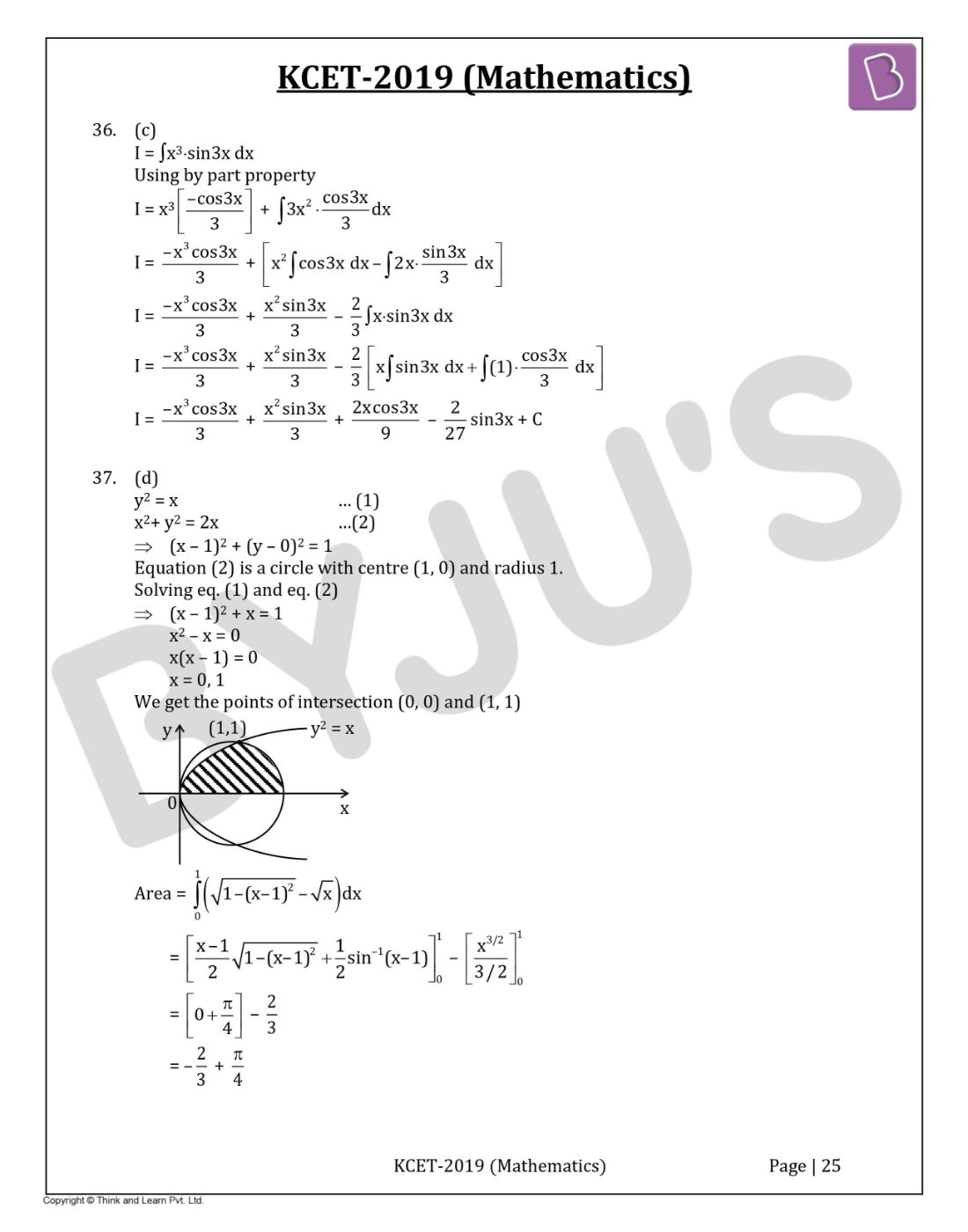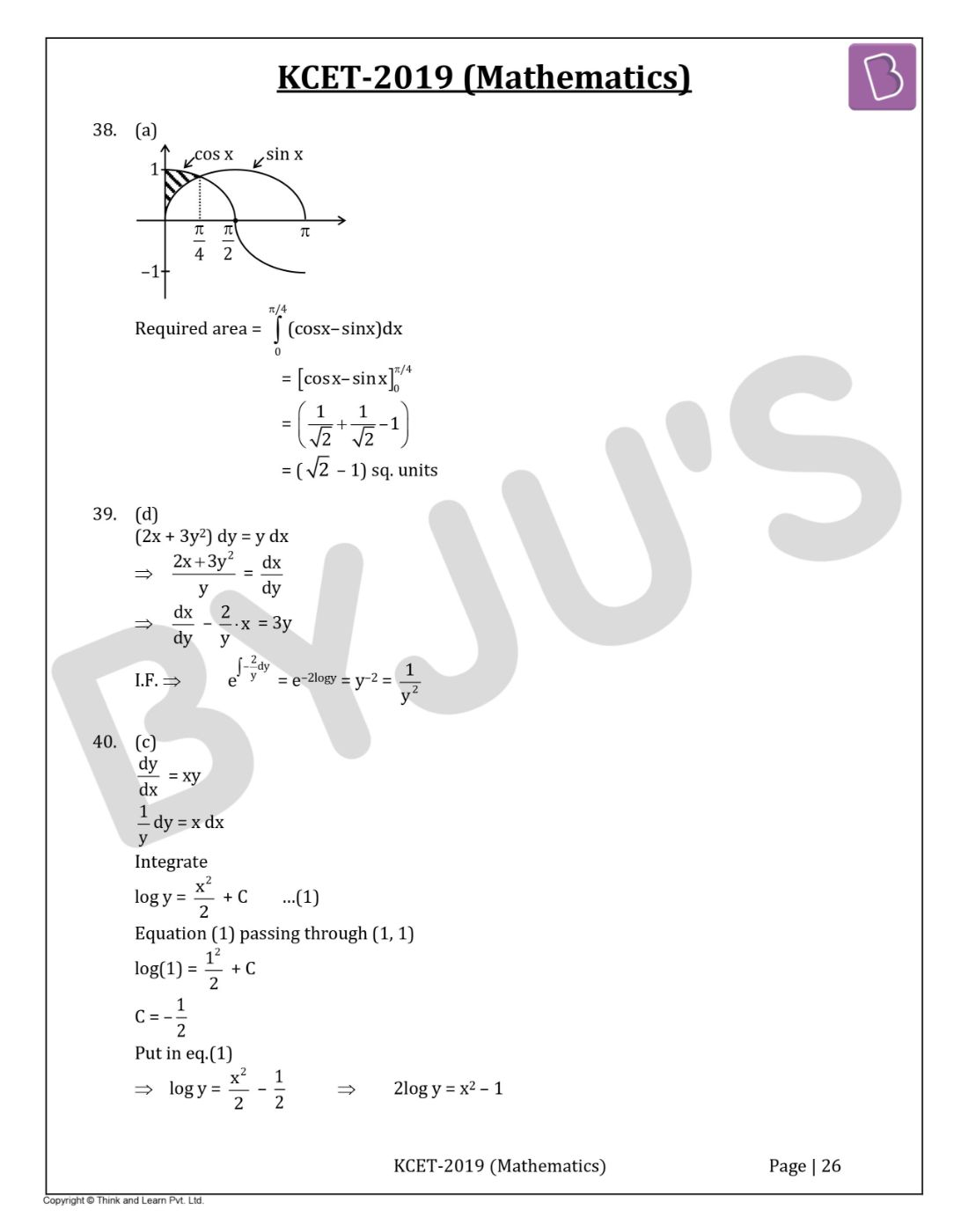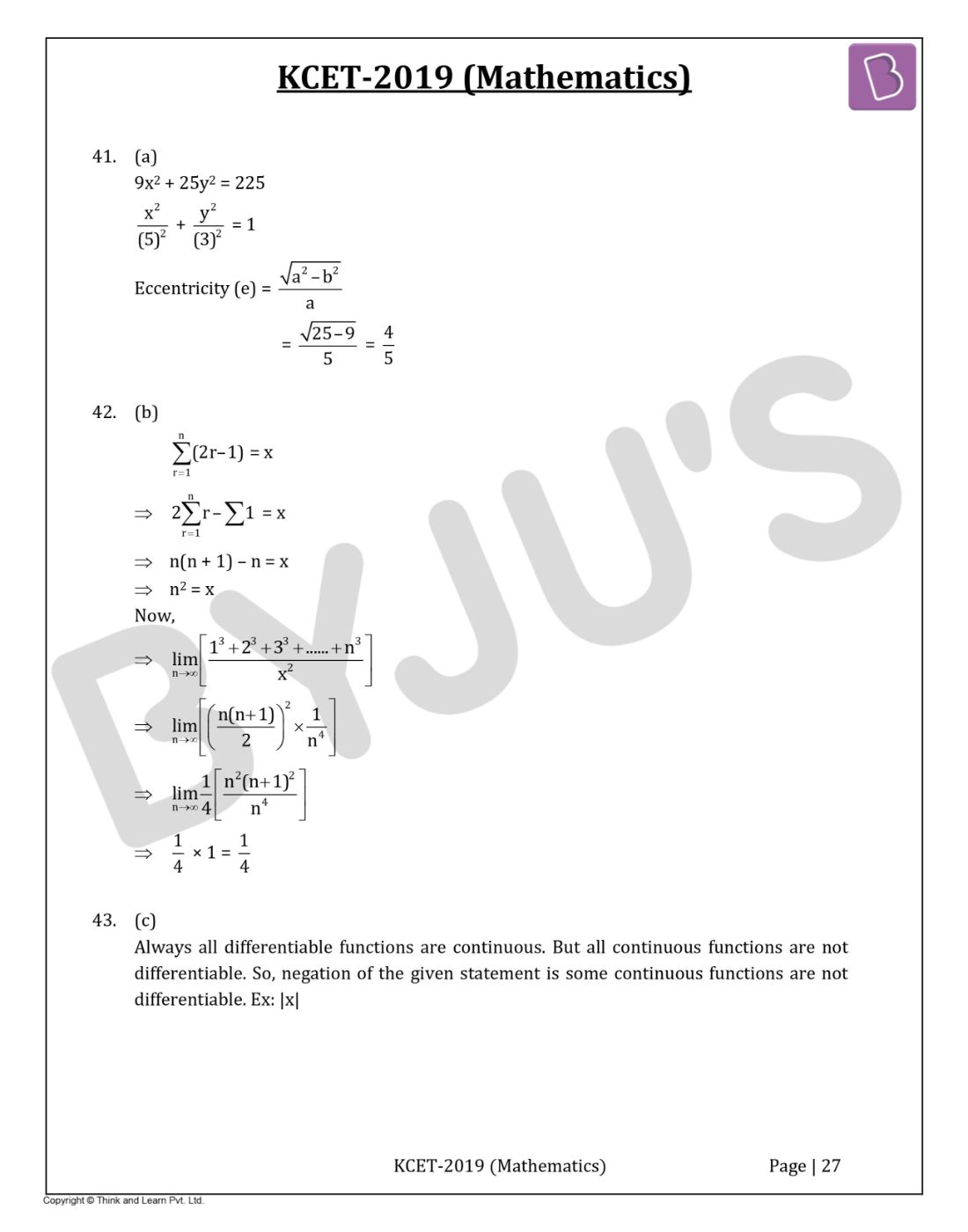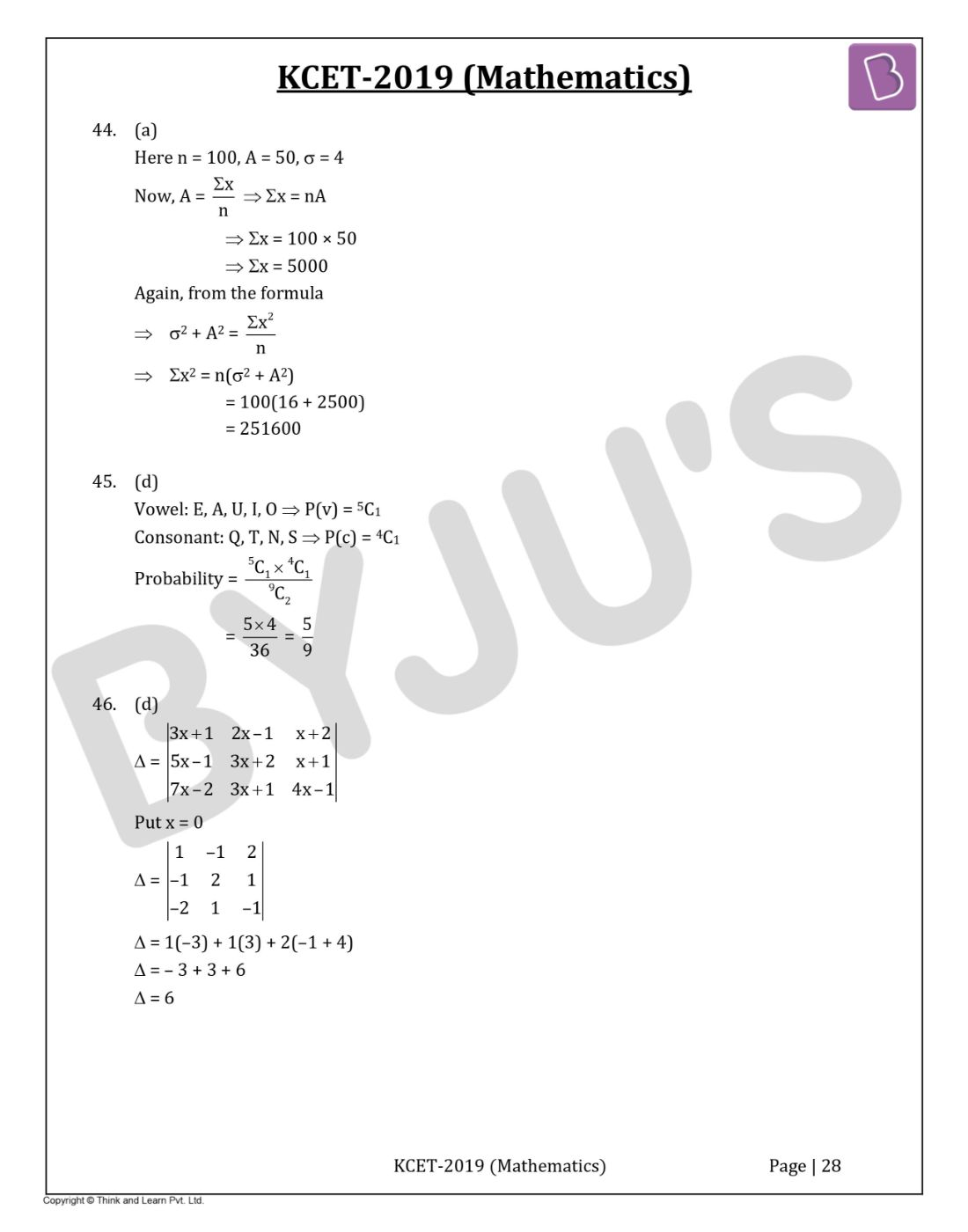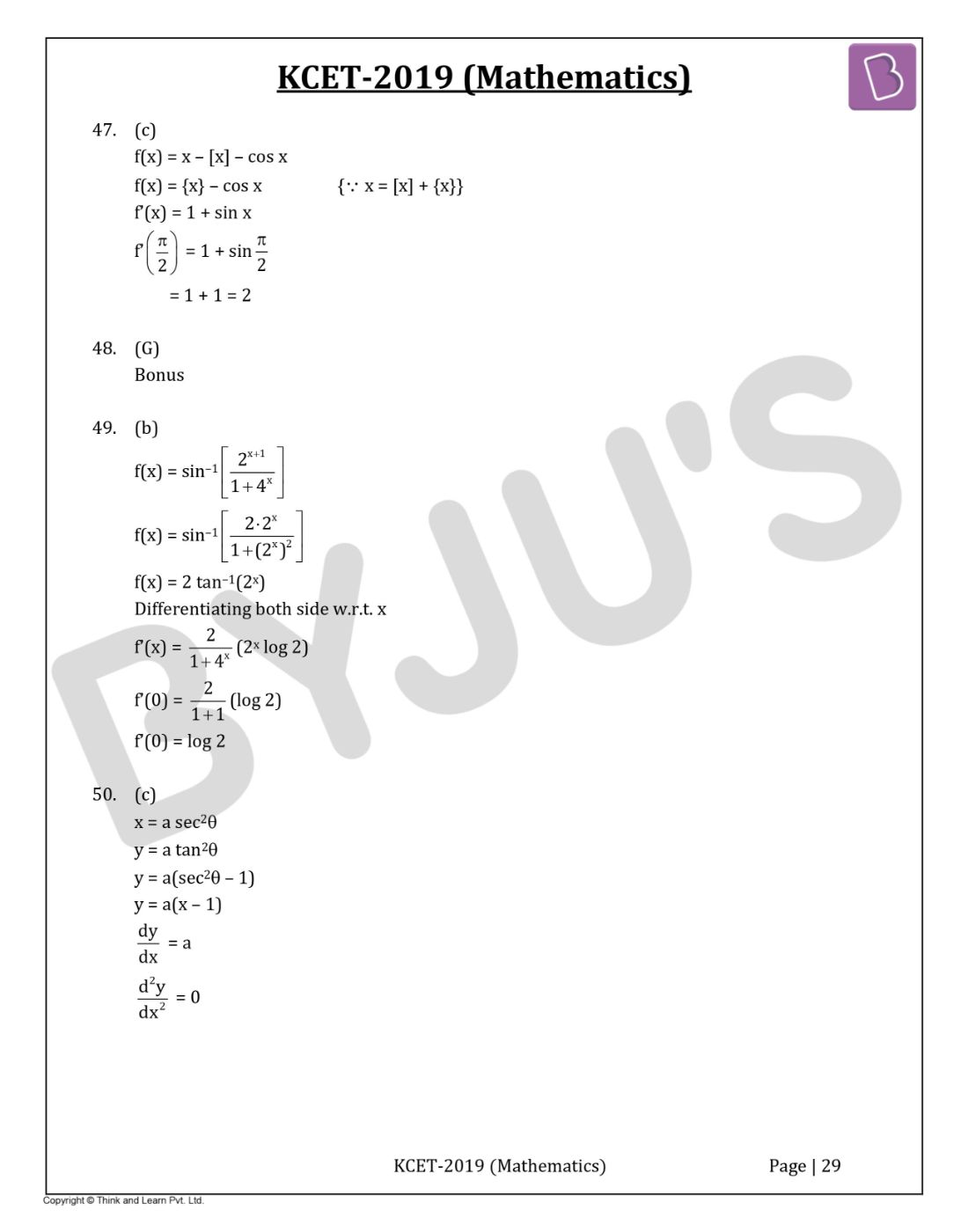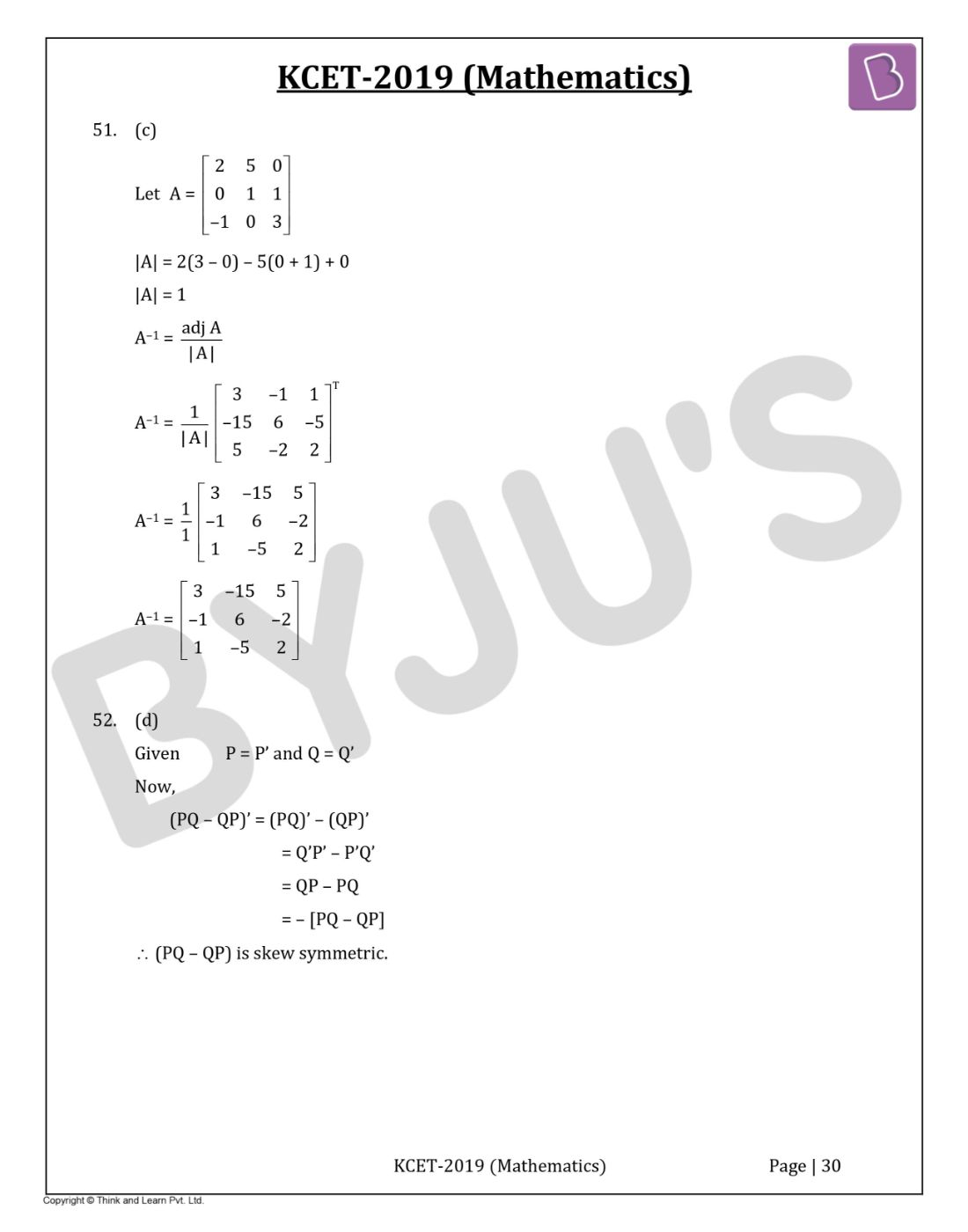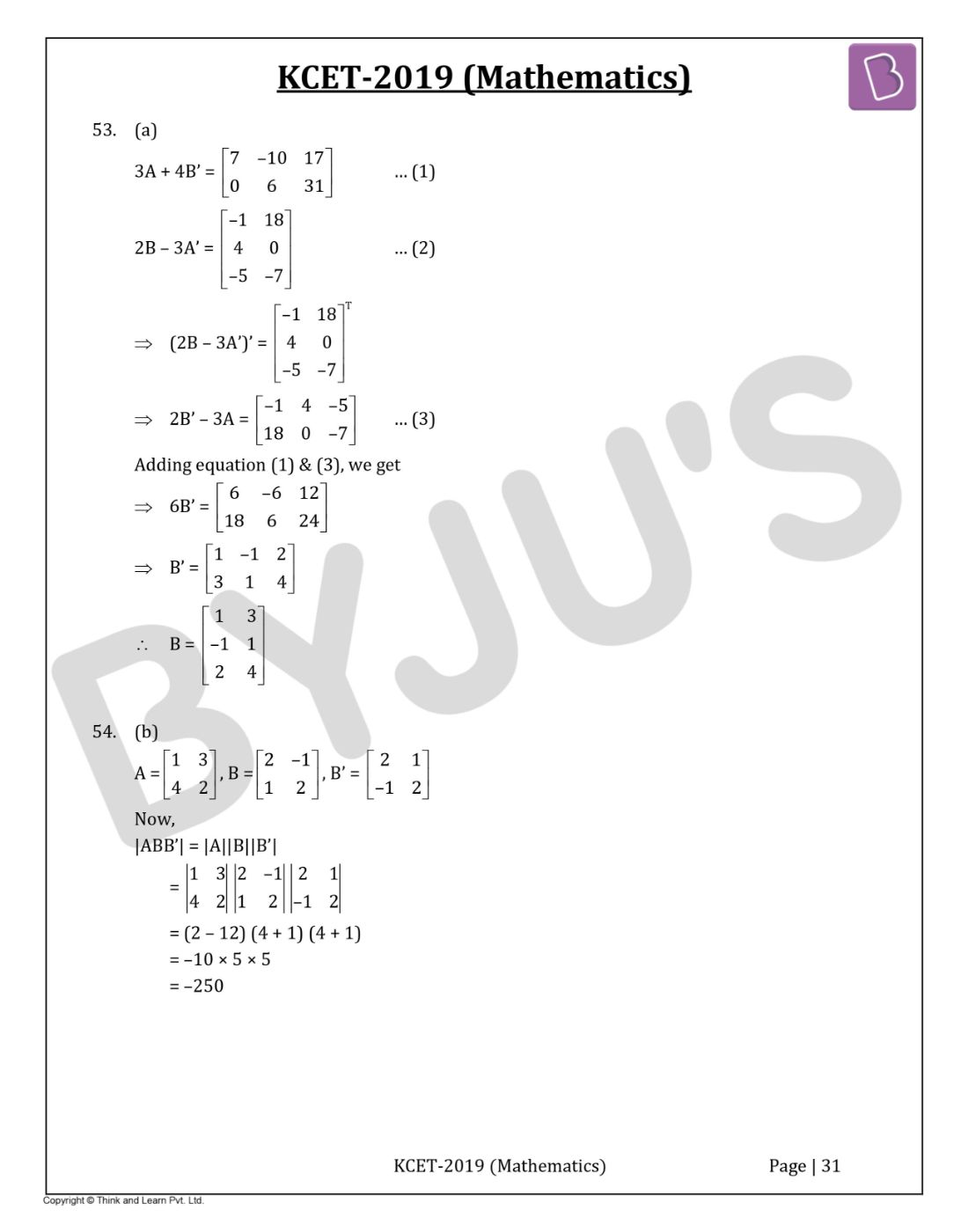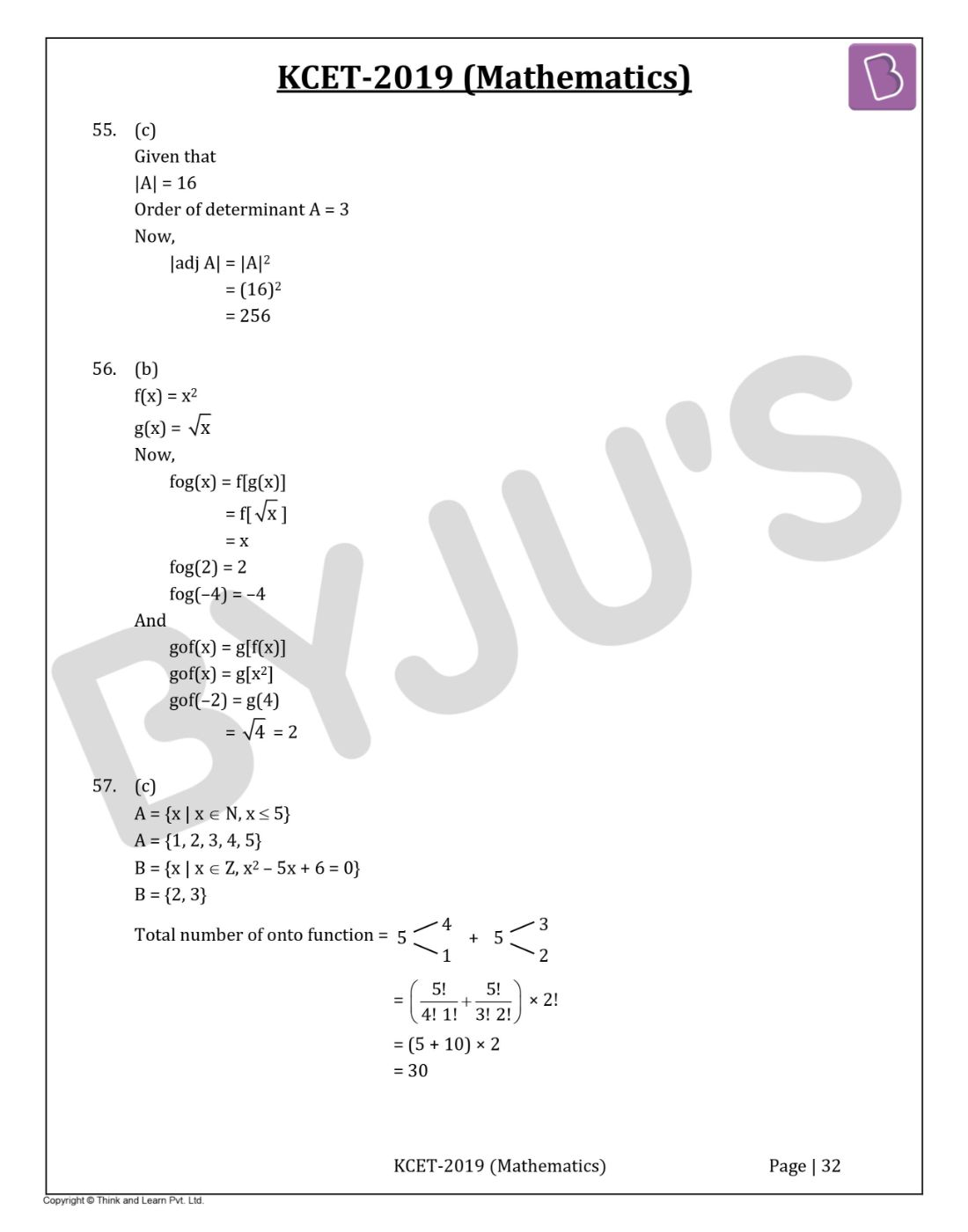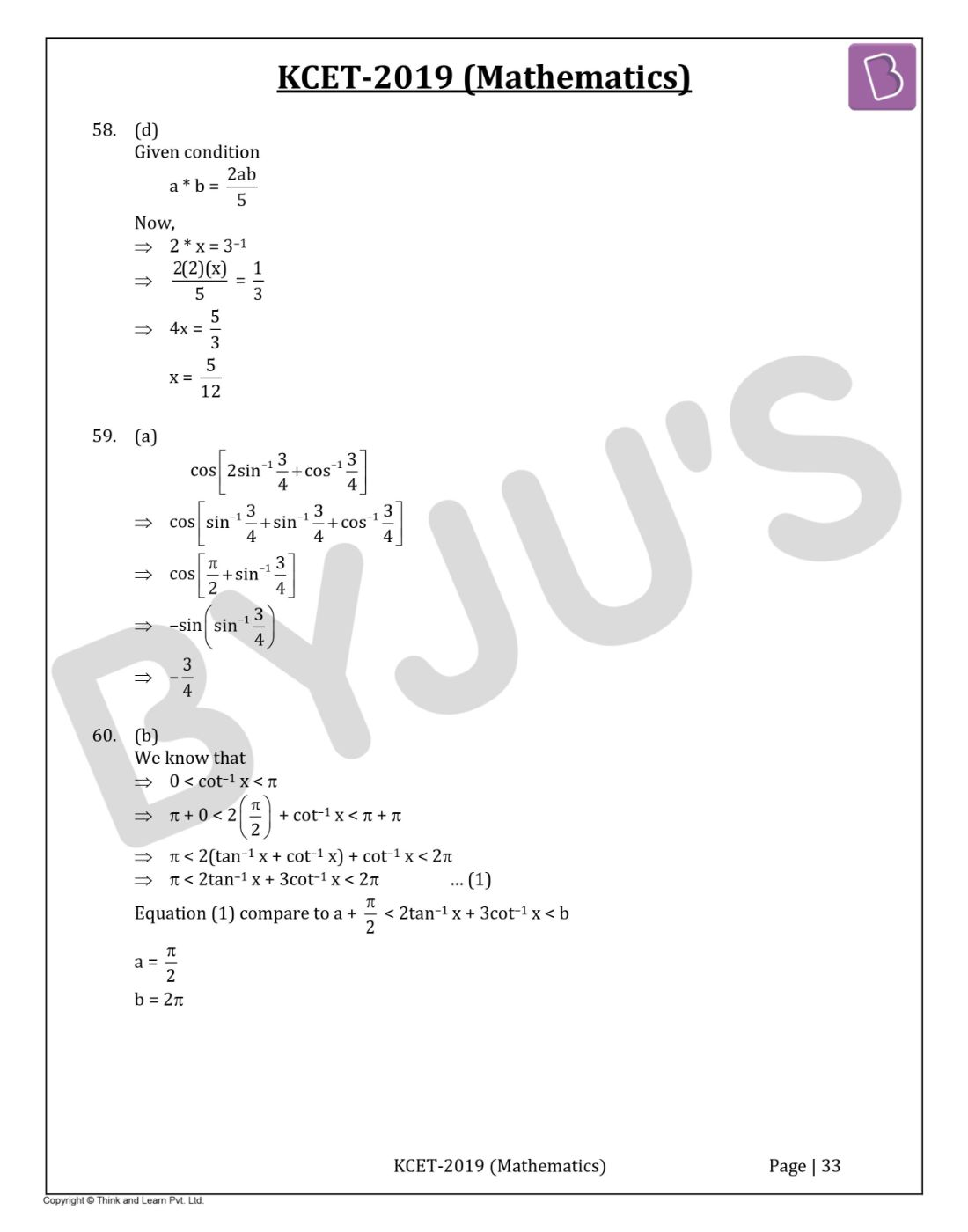1. Need question papers

2. very useful

3. I need KCET important pyq’s for 2023 exam

4. I HAVE SOME QUETION PAPERS FOR PREPRATION FOR KCET EXAMS

5. Good

6. This app is very usful and I need the question paper for pyq kcet maths

7. Very use full

8. Who is theory of relativity

9. I want kcet test papers

10. It is very useful

11. Super solution
Thanks